• 第一章 C游戏开发快速入门 学习本章前需要掌握的语法知识：标识符、变量、常量、运算符与表达式，以及printf、scanf、if-else、while、for语句的用法 原书中环境VS2010 1.1弹跳球小球 #include <stdio.h> #...

第一章 C游戏开发快速入门

• 学习本章前需要掌握的语法知识：标识符、变量、常量、运算符与表达式，以及printf、scanf、if-else、while、for语句的用法
• 原书中环境VS2010
• 随书资源[密：cq2g]

1.1弹跳球小球

#include <stdio.h>
#include <stdlib.h>
#include <Windows.h>

int main(void)
{
int i, j;
int x = 0;
int y = 5;

int height = 20;
int velocity_x = 1;
int velocity_y = 1;
int left = 0;
int right = 20;
int top = 0;
int bottom = 10;

while ( 1 )
{
x += velocity_x;
y += velocity_y;
system("cls");				// 清屏函数
// 输出小球上面的空行
for ( i = 0; i < x; i++ )
printf("\n");

// 输出小球左边的空行
for ( j = 0; j < y; j++ )
printf(" ");

printf("o");				// 输出小球o
printf("\n");
Sleep(50);					// 在输出图形后等待50ms

if ( x == top || x == bottom )
velocity_x = -velocity_x;
if ( y == left || y == right )
velocity_y = -velocity_y;
}

return 0;
}

思考题

1.如果不用Sleep函数，能否利用循环语句实现速度变慢的效果？

2.尝试利用printf("\a")实现小球碰撞到边界时响铃的效果。

3.尝试为反弹球游戏绘制边框。

1.2 简单的飞机游戏

#include <stdio.h>
#include <stdlib.h>
#include <conio.h>						// 输入函数：getch()、kbhit()

int main(void)
{
int i, j;
int x = 5;
int y = 10;
char input;
int isFire = 0;						// 1显示飞机在正上方输出激光竖线

int ny = 5;							// 第一个靶子，放在第一行的ny列上
int isKilled = 0;
// 0显示靶子，1靶子被击中则不显示

while ( 1 )
{
system("cls");					// 清屏函数

if ( !isKilled )				// 输出靶子
{
for ( j = 0; j < ny; j++ )
printf(" ");
printf("+ \n");
}

if ( isFire == 0 )				// 输出飞机上面的空行
{
for ( i = 0; i < x; i++ )
printf("\n");
}else
{
for ( i = 0; i < x; i++ )	// 输出飞机上面的激光光线
{
for ( j = 0; j < y; j++ )
printf(" ");
printf("  |\n");
}
if ( ny == y + 2 )			// +2 是因为激光在飞机的正中间，距离左边的而坐标
isKilled = 1;			// 击中靶子
isFire = 0;
}
// 下面输出一个复杂的飞机图案
for ( j = 0; j < y; j++ )
printf(" ");
printf("  * \n");				// 输出飞机
for ( j = 0; j < y; j++ )
printf(" ");
printf("*****\n");
for ( j = 0; j < y; j++ )
printf(" ");
printf(" * * \n");

if ( kbhit() )					// 判断是否有输入
{
input = getch();			// 根据用户的不同输入来移动，不必输入回车

if ( input == 'a' )
y--;					// 位置左移
if ( input == 'd' )
y++;					// 位置右移
if ( input == 'w' )
x--;					// 位置上移
if ( input == 's' )
x++;					// 位置下移
if ( input == ' ' )
isFire = 1;

}
}

return 0;
}

思考题

1.如何让靶子移动起来。
2.如何统计和显示击中得分。

总结

1、大框架：建立（x，y）坐标【行、列】建立循环体。
2、循环体作用域中使用if else语句创建图案。
3、对于不同的图案可使用标记变量进行操作判断。
（如果这个点需要移动则放在大框内，否则放在框架外）

//以下函数只能在windows os使用
#include <stdlib.h>		// 提供system("cls")；清屏函数
#include <windows.h>	// 提供Sleep(50);睡眠50ms
#include <conio.h>
/*
提供：	输入函数：getch()，不需要回车就可以得到输入行的字符。
kbhit()函数在用户有键盘输入时，返回1，否则返回0；

*/

第二章 函数封装的游戏开发

2.1 飞机游戏

#include <stdio.h>
#include <stdlib.h>						// 提供 system("cls");	清屏函数
#include <conio.h>						// 提供 getch()、kbhit() 函数
#include <Windows.h>					// 提供 void gotoxy(int x, int y); 函数

// 全局变量
int position_x, position_y;				// 飞机位置
int bullet_x, bullet_y;					// 子弹位置
int enemy_x, enemy_y;					// 敌机位置
int high, width;						// 游戏画面尺寸
int score;								// 得分

void startup()							// 数据初始化
{
score = 0;
high = 20;
width = 30;
position_x = high/2;
position_y = width/2;
bullet_x = -1;
bullet_y = position_y;
enemy_x = 0;
enemy_y = position_y;
}

void gotoxy(int x, int y)				/* 将光标移动到(x,y)位置 */
{
HANDLE handle = GetStdHandle(STD_OUTPUT_HANDLE);
COORD pos;
pos.X = x;
pos.Y = y;
SetConsoleCursorPosition(handle, pos);
}

void show()								/* 展示画面 */
{
//system("cls");					// 清屏
gotoxy(0,0);						// 光标移动到原点位置，以下重画清屏
int i, j;
for ( i = 0; i < high; i++ )
{
for ( j = 0; j < width; j++ )
{
if ( (i == position_x) && (j == position_y) )
printf("*");			// 输出飞机*
else if ( (i == enemy_x) && (j == enemy_y) )
printf("@");			// 输出敌机@
else if( (i == bullet_x) && (j == bullet_y) )
printf("|");			// 输出子弹|
else
printf(" ");			// 输出空格
}
printf("\n");
}
printf("得分：%d\n", score);
}

void updateWithoutInput()				/* 与用户输入无关的更新 */
{
if ( bullet_x > -1 )
bullet_x--;

// 用来控制敌机向下移动的速度，每隔几次循环才移动一次敌机
// 这样修改，虽然用户按键的交互速度还是很快，但 NPC 的移动显示可以降速
static int speed = 0;
if ( speed < 10 )
{
speed++;
}
if ( speed == 10 )
{
enemy_x++;
speed = 0;
}

if ( (bullet_x == enemy_x) && (bullet_y == enemy_y) )	//子弹击中敌机
{
score++;
enemy_x = -1;					// 产生新的飞机
enemy_y = rand() % width;
bullet_x = -2;					// 子弹无效(防止穿射)【等于0以下均可，这里只是用-2标识被击落】
}

if ( enemy_x > high )				// 敌机抛出显示屏幕
{
enemy_x = -1;					// 产生新的飞机
enemy_y = rand() % width;
}

}

void updateWithInput()					/* 与用户输入有关的更新 */
{
char input;
if (kbhit())						// 判断是否有输入
{
input = getch();				// 根据用户的不同输入来移动，不必输入回车
if ( input == 'a' )
position_y--;				// 位置左移
if ( input == 'd' )
position_y++;				// 位置右移
if ( input == 'w' )
position_x--;				// 位置上移
if ( input == 's' )
position_x++;				// 位置下移
if ( input == ' ' )
{
bullet_x = position_x - 1;	// 发射子弹的初始位置在飞机的正上方
bullet_y = position_y;
}
}
}

int main()
{
startup();						// 数据的初始化
while ( 1 )						// 游戏循环执行
{
show();						// 显示画面
updateWithoutInput();		// 与用户输入无关的更新
updateWithInput();			// 与用户输入有关的更新
}

return 0;
}

思考题：

1.参考1.2.3节中的方法，尝试实现复杂的飞机图形。
2.随着积分的增加加快敌机的下落速度。
3.防止玩家操控飞机飞出边界。
4.增加按Esc键后暂停的功能。

2.2 用函数实现反弹球消砖块

#include <stdio.h>
#include <stdlib.h>
#include <conio.h>
#include <windows.h>

// 全局变量
int high, width;					// 游戏画面大小
int ball_x, ball_y;					// 小球的坐标
int ball_vx, ball_vy;				// 小球的速度
int position_x, position_y;			// 挡板的中心坐标
int ridus;							// 挡板的半径大小
int left, right;					// 挡板的左右位置
int ball_number;					// 反弹小球的次数
int block_x, block_y;				// 砖块的位置
int score;							// 消除砖块的个数

void gotoxy(int x, int y)			/* 将光标移动到(x, y)位置 */
{
HANDLE handle = GetStdHandle(STD_OUTPUT_HANDLE);
COORD pos;
pos.X = x;
pos.Y = y;
SetConsoleCursorPosition(handle, pos);
}

void startup()						/* 数据初始化 */
{
high = 15;
width = 20;
ball_x = 0;
ball_y = width / 2;
ball_vx = 1;
ball_vy = 1;
ridus = 5;
position_x = high;
position_y = width / 2;
left = position_y - ridus;
right = position_y + ridus;
ball_number = 0;
block_x = 0;
block_y = width / 2 + 1;
score = 0;
}

void show()							/* 显示画面 */
{
gotoxy(0, 0);					// 光标移动到原点位置，以下重画清屏
int i, j;
for ( i = 0; i <= high + 1; i++ )
{
for ( j = 0; j <= width; j++ )
{
if ( (i == ball_x) && (j == ball_y) )
printf("0");		// 输出小球
else if ( j == width )
printf("|");		// 输出右边框
else if ( i == high + 1 )
printf("-");		// 输出下边框
else if ( (i == position_x) && (j >= left) && (j <= right) )
printf("*");		// 输出挡板
else if ( (i == block_x) && (j == block_y) )
printf("B");
else
printf(" ");		// 输出空格
}
printf("\n");
}
printf("反弹小球数:%d\n", ball_number);
printf("消除的砖块数:%d\n", score);
}

void updateWithoutInput()			/* 与用户输入无关的更新 */
{
if ( ball_x == high - 1 )
{
if ( (ball_y >= left) && (ball_y <= right) )			// 被挡板挡住
{
ball_number++;
printf("\a");
}else
{
printf("游戏失败\n");
system("pause");
exit(EXIT_FAILURE);
}
}

if ( (ball_x == block_x) && (ball_y == block_y) )
{
score++;					// 分数加1
block_y = rand() % width;	// 产生新的砖块
}

ball_x = ball_x + ball_vx;
ball_y = ball_y + ball_vy;
if ( (ball_x == 0) || (ball_x == high - 1) )
ball_vx = -ball_vx;
if ( (ball_y == 0) || (ball_y == width - 1) )
ball_vy = -ball_vy;

Sleep(80);
}

void updateWithInput()				/* 与用户输入有关的更新 */
{
char input;
if ( kbhit() )					// 判断是否有输入
{
input = getch();			// 根据用户的不同输入来移动，不必输入回车
if ( input == 'a' )
{
position_y--;			// 位置左移
left = position_y - ridus;
right = position_y + ridus;
}
if ( input == 'd' )
{
position_y++;			// 位置右移
left = position_y - ridus;
right = position_y + ridus;
}
}
}

int main(void)
{
startup();						// 游戏的初始化
while ( 1 )
{
show();						// 显示画面
updateWithoutInput();		// 与用户输入无关的更新
updateWithInput();			// 与用户输入有关的更新
}

return 0;
}

思考题

1、增加砖块，使得集中概率增大。
2、实现对小球更多的操控，从而可以调整击中的砖块。

2.3 flappy bird

#include <stdio.h>
#include <stdlib.h>
#include <Windows.h>
#include <conio.h>

// 全局变量
int high, width;						// 游戏画面大小
int bird_x, bird_y;						// 小鸟的坐标
int bar1_y, bar1_xDown, bar1_xTop;		// 障碍物
int score;								// 得分，经过障碍物的个数

void gotoxy(int x, int y)				/* 将光标移动到(x, y)位置 */
{
HANDLE handle = GetStdHandle(STD_OUTPUT_HANDLE);
COORD pos;
pos.X = x;
pos.Y = y;
SetConsoleCursorPosition(handle, pos);
}

void startup()							/* 数据的初始化 */
{
high = 20;
width = 59;
bird_x = 0;
bird_y = width / 3;
bar1_y = width / 2;
bar1_xDown = high / 3;
bar1_xTop = high / 2;
score = 0;
}

void show()								/* 显示画面 */
{
gotoxy(0,0);						// 光标移动到原点位置，以下重画清屏
int i, j;

for ( i = 0; i < high; i++ )
{
for ( j = 0; j < width; j ++ )
{
if ( (i == bird_x) && (j == bird_y) )
printf("@");			// 输出小鸟
else if ( (j == bar1_y) && ((i < bar1_xDown) || (i > bar1_xTop)) )
printf("*");
else
printf(" ");			// 输出空格
}
printf("\n");
}
printf("得分：%d\n", score);
}

void updateWithoutInut()				/* 与用户无关的更新 */
{
bird_x++;
bar1_y--;

if ( (bird_y == bar1_y) )
{
if ( (bird_x >= bar1_xDown) && (bird_x <= bar1_xTop) )
score++;
else
{
printf("游戏失败\n");
system("pause");
exit(EXIT_FAILURE);
}
}
if ( bar1_y <= 0 )					//重新生成新的障碍物
{
bar1_y = width;
int temp = rand() % (int)(high * 0.8);
bar1_xDown = temp - high / 10;
bar1_xTop = temp + high / 10;
}

Sleep(150);
}

void updateWithInput()
{
char input;
if ( kbhit() )					// 判断是否有输入
{
input = getch();			// 根据用户的不同输入来移动，不必输入回车
if ( input == ' ' )
{
bird_x = bird_x - 2;
}
}
}

int main(void)
{
startup();
while ( 1 )
{
show();						// 显示画面
updateWithoutInut();		// 与用户输入无关的更新
updateWithInput();			// 与用户输入有关的更新
}

return 0;
}

思考题

1、实现小鸟受重力影响下落的效果，即加速度
2、模拟原版flappy bird游戏，在游戏画面中同时显示多个柱子。

总结

#include <Windows.h>					// 提供 void gotoxy(int x, int y); 函数

void gotoxy(int x, int y)				/* 将光标移动到(x,y)位置 */
{
HANDLE handle = GetStdHandle(STD_OUTPUT_HANDLE);
COORD pos;
pos.X = x;
pos.Y = y;
SetConsoleCursorPosition(handle, pos);
}// 替代system("cls")清屏函数

// 固定句型
int main(void)
{
startup();
while ( 1 )
{
show();						// 显示画面
updateWithoutInut();		// 与用户输入无关的更新
updateWithInput();			// 与用户输入有关的更新
}

return 0;
}

// 结束程序语句
{
printf("游戏失败\n");
system("pause");
exit(EXIT_FAILURE);
}

第三章 应用数组的游戏开发

3.1 生命游戏

#include <stdio.h>
#include <stdlib.h>
#include <conio.h>
#include <Windows.h>
#include <time.h>

#define HIGH 25							// 游戏画面尺寸
#define WIDTH 50

//全局变量
int cells[HIGH][WIDTH];					// 所有位置细胞生1或死0

void gotoxy(int x, int y)				/* 将光标移动到(x,y)位置 */
{
HANDLE handle = GetStdHandle(STD_OUTPUT_HANDLE);
COORD pos;
pos.X = x;
pos.Y = y;
SetConsoleCursorPosition(handle, pos);
}// 替代system("cls")清屏函数

void startup()
{
int i, j;
for ( i = 0; i < HIGH; i++ )
{
for ( j = 0; j < WIDTH; j++ )
{
cells[i][j] = 1;
}
}
}

void show()							/* 显示画面 */
{
gotoxy(0,0);						// 光标移动到原点位置，以下重画清屏
int i, j;
for ( i = 0; i < HIGH; i++ )
{
for ( j = 0; j < WIDTH; j++ )
{
if ( cells[i][j] == 1 )
printf("*");			// 输出活的细胞
else
printf(" ");			// 输出空格
}
printf("\n");
}
Sleep(50);
}

void updateWithoutInput()				/* 与用户输入无关的更新 */
{
int NewCells[HIGH][WIDTH];			// 下一帧的细胞情况
int NeibourNumber;					// 统计邻居的个数
int i, j;
for ( i = 1; i <= HIGH - 1; i++ )
{
for ( j = 1; j <= WIDTH - 1; j++ )
{
NeibourNumber = cells[i - 1][j - 1] + cells[i - 1][j] + cells[i - 1][j + 1]
+cells[i][j - 1] + cells[i][j + 1] + cells[i + 1][j - 1] + cells[i + 1][j]
+ cells[i + 1][j + 1];

if ( NeibourNumber == 3 )
NewCells[i][j] = 1;
else if ( NeibourNumber == 2 )
NewCells[i][j] == cells[i][j];
else
NewCells[i][j] = 0;
}
}

for ( i = 1; i <= HIGH - 1; i++ )
{
for ( j = 1; j <= WIDTH - 1; j++ )
cells[i][j] = NewCells[i][j];
}
}

void updateWithInput()
{

}

int main(void)
{
startup();

while ( 1 )
{
show();
updateWithoutInput();
updateWithInput();
}

return 0;
}

思考题

1、让某块区域有水源，即在某块区域声明更容易生存、繁殖
2、实现按+键生命游戏加速演化显示，-键减速，Esc键暂停、R键重新开始
3、实现捕食者、猎物组成的生命游戏，分别用不同的字符显示。

3.2 用数组实现反弹球小砖块

#include <stdio.h>
#include <stdlib.h>
#include <Windows.h>
#include <conio.h>

#define HIGH 15						// 游戏画面尺寸
#define WIDTH 20

// 全局变量
int ball_x, ball_y;					// 小球的坐标
int ball_vx, ball_vy;				// 小球的速度
int position_x, position_y;			// 挡板的中心坐标
int left, right;					// 挡板的左右位置
int canvas[HIGH][WIDTH] = { 0 };	// 二维数组存储游戏画布中对应的元素
// 0为空格，1为小球o，2为挡板,3为砖块#

void gotoxy(int x, int y)				/* 将光标移动到(x,y)位置 */
{
HANDLE handle = GetStdHandle(STD_OUTPUT_HANDLE);
COORD pos;
pos.X = x;
pos.Y = y;
SetConsoleCursorPosition(handle, pos);
}// 替代system("cls")清屏函数

void startup()
{
ball_x = 0;
ball_y = WIDTH / 2;
ball_vx = 1;
ball_vy = 1;
canvas[ball_x][ball_y] = 1;

position_x = HIGH - 1;
position_y = WIDTH / 2;

int k, i;
for ( k = left; k <= right; k++ )
canvas[position_x][k] = 2;

for ( k = 0; k < WIDTH; k++ )
{
for ( i = 0; i < HIGH / 4; i++ )
canvas[i][k] = 3;
}
}

void show()
{
gotoxy(0,0);
int i, j;

for ( i = 0; i < HIGH; i++ )
{
for ( j = 0; j < WIDTH; j++ )
{
if ( canvas[i][j] == 0 )
printf(" ");				// 输出空格
else if ( canvas[i][j] == 1 )
printf("o");				// 输出小球o
else if ( canvas[i][j] == 2 )
printf("*");				// 输出挡板
else if ( canvas[i][j] == 3 )
printf("#");				// 输出砖块#
}
printf("|\n");						// 显示右边界
}
for( j = 0; j < WIDTH; j++ )
printf("-");						// 显示下边界
printf("\n");
}

void updateWithoutInput()					/* 与用户输入无关的更新 */
{
if ( ball_x == HIGH - 2 )
{
if ( (ball_y >= left) && (ball_y <= right) )
printf("\a");					// 被挡板挡住响铃
else								// 没有被挡板挡住
{
printf("游戏失败\n");
system("pause");
exit(EXIT_FAILURE);
}
}

static int speed = 0;
if ( speed < 7 )
speed++;

if ( speed == 7 )
{
speed = 0;

canvas[ball_x][ball_y] = 0;
// 更新小球的坐标
ball_x = ball_x + ball_vx;
ball_y = ball_y + ball_vy;
canvas[ball_x][ball_y] = 1;

// 碰到边界后反弹
if ( (ball_x == 0) || (ball_x == HIGH - 2) )
ball_vx = -ball_vx;
if ( (ball_y == 0) || (ball_y == WIDTH - 1) )
ball_vy = -ball_vy;

// 碰到砖块后反弹
if ( canvas[ball_x - 1][ball_y] == 3 )
{
ball_vx = -ball_vx;
canvas[ball_x - 1][ball_y] = 0;
printf("\a");
}
}
}

void updateWithInput()						/* 与用户输入无关的更新 */
{
char input;
if ( kbhit() )							// 判断是否有输入
{
input = getch();					// 根据用户的不同输入来移动，不必输入回车
if ( input == 'a' && left > 0)
{
canvas[position_x][right] = 0;
position_y--;					// 位置左移
canvas[position_x][left] = 2;
}
if ( input == 'd' && right < WIDTH - 1 )
{
canvas[position_x][left] = 0;
position_y++;					// 位置右移
canvas[position_x][right] = 2;
}
}
}

int main(void)
{
startup();								// 数据的初始化
while ( 1 )								// 游戏循环执行
{
show();								// 显示画面
updateWithoutInput();				// 与用户输入无关的更新
updateWithInput();					// 与用户输入有关的更新
}

return 0;
}

思考题

1、按空格键发射新的小球。
2、尝试接金币的小游戏。

3.3 空战游戏

#include <stdio.h>
#include <stdlib.h>
#include <conio.h>
#include <Windows.h>

#define HIGH 25								// 游戏画面尺寸
#define WIDTH 50
#define EnemyNum 5

// 全局变量
int position_x, position_y;					// 飞机的位置
int enemy_x[EnemyNum], enemy_y[EnemyNum];	// 敌机的位置
int canvas[HIGH][WIDTH] = {0};				// 二位数组存储游戏画布中对应的元素
// 0为空格，1为飞机， 2为子弹|， 3为敌机@
int score;
int BulletWidth;							// 子弹的宽度
int EnemyMoveSpeed;							// 敌机的移动速度

void gotoxy(int x, int y)					/* 将光标移动到(x,y)位置 */
{
HANDLE handle = GetStdHandle(STD_OUTPUT_HANDLE);
COORD pos;
pos.X = x;
pos.Y = y;
SetConsoleCursorPosition(handle, pos);
}// 替代system("cls")清屏函数

void startup()								/* 数据的初始化 */
{
position_x = HIGH / 2;
position_y = WIDTH / 2;
canvas[position_x][position_y] = 1;

int k;
for ( k = 0; k < EnemyNum; k++ )
{
enemy_x[k] = rand() % 2;
enemy_y[k] = rand() % WIDTH;
canvas[enemy_x[k]][enemy_y[k]] = 3;
}
score = 0;
BulletWidth = 0;
EnemyMoveSpeed = 20;
}

void show()									// 显示画面
{
gotoxy(0,0);							// 光标移动到原点位置，以下重画清屏
int i, j;

for ( i = 0; i < HIGH; i++ )
{
for ( j = 0; j < WIDTH; j++ )
{
if ( canvas[i][j] == 0 )
printf(" ");				// 输出空格
else if ( canvas[i][j] == 1 )
printf("*");				// 输出飞机*
else if ( canvas[i][j] == 2 )
printf("|");				// 输出子弹|
else if ( canvas[i][j] == 3 )
printf("@");				// 输出敌机@
}
printf("\n");
}
printf("得分：%3d\n", score);
//Sleep(20);
}

void updateWithoutInput()					/* 与用户输入无关的更新 */
{
int i, j, k;
for ( i = 0; i < HIGH; i++ )
{
for ( j = 0; j < WIDTH; j++ )
{
if ( canvas[i][j] == 2 )									// 子弹向上移动
{
for ( k = 0; k < EnemyNum; k++ )
{
if ( (i == enemy_x[k]) && (j == enemy_y[k]) )		// 子弹击中敌机
{
score++;										// 分数加1
if ( score % 5 == 0 && EnemyMoveSpeed > 3 )		// 达到移动积分后敌机变快
EnemyMoveSpeed--;
if ( score % 5 == 0 )							// 达到一定积分后子弹变厉害
BulletWidth++;
canvas[enemy_x[k]][enemy_y[k]] = 0;
enemy_x[k] = rand() % 2;						// 产生新的飞机
enemy_y[k] = rand() % WIDTH;
canvas[enemy_x[k]][enemy_y[k]] = 3;
canvas[i][j] = 0;								// 子弹消失
}
}

// 子弹向上移动
canvas[i][j] = 0;
if ( i > 0 )
canvas[i - 1][j] = 2;
}
}
}

static int speed = 0;
if ( speed < EnemyMoveSpeed )
speed++;

for ( k = 0; k < EnemyNum; k++ )
{
if ( (position_x == enemy_x[k]) && (position_y == enemy_y[k]) )					// 敌机撞到我机
{
printf("失败!\n");
Sleep(3000);
system("pause");
exit(EXIT_FAILURE);
}

if ( enemy_x[k] > HIGH )				// 敌机抛出显示屏幕
{
canvas[enemy_x[k]][enemy_y[k]] = 0;
enemy_x[k] = rand() % 2;
enemy_y[k] = rand() % WIDTH;
canvas[enemy_x[k]][enemy_y[k]] = 3;
score--;							// 减分
}

if ( speed == EnemyMoveSpeed )
{
// 敌机下落
for ( k = 0; k < EnemyNum; k++ )	// 这里最好再定义一个变量，为了方便就不定义了
{
canvas[enemy_x[k]][enemy_y[k]] = 0;
enemy_x[k]++;
canvas[enemy_x[k]][enemy_y[k]] = 3;
speed = 0;
}
}
}
}

void updateWithInput()							/* 与用户输入有关的更新 */
{
int k;
char input;
if ( kbhit() )								// 判断是否有输入
{
input = getch();						// 根据用户的不同输入来移动，不必输入回车
if ( input == 'a' )
{
canvas[position_x][position_y] = 0;
position_y--;						// 位置左移
canvas[position_x][position_y] = 1;
}else if ( input == 'd' )
{
canvas[position_x][position_y] = 0;
position_y++;						// 位置右移
canvas[position_x][position_y] = 1;
}else if ( input == 'w' )
{
canvas[position_x][position_y] = 0;
position_x--;						// 位置上移
canvas[position_x][position_y] = 1;
}else if ( input == 's' )
{
canvas[position_x][position_y] = 0;
position_x++;						// 位置下移
canvas[position_x][position_y] = 1;
}else if ( input == ' ' )
{
int left = position_y - BulletWidth;
int right = position_y + BulletWidth;
if ( left < 0 )
left = 0;
if ( right > WIDTH - 1)
right = WIDTH - 1;

for ( k = left; k <= right; k++ )	// 发射子弹
canvas[position_x - 1][k] = 2;	// 发射子弹的初始化位置再飞机的正上方
}
}
}

int main(void)
{
startup();									// 游戏的初始化
while ( 1 )									// 游戏循环执行
{
show();									// 显示画面
updateWithoutInput();					// 与用户输入无关的更新
updateWithInput();						// 与用户输入有关的更新
}

return 0;
}

思考题

1、增加敌机BOSS，其形状更大、血量更多。
2、尝试让游戏更有趣，敌机也发射子弹。

3.4 贪吃蛇

#include <stdio.h>
#include <stdlib.h>
#include <conio.h>
#include <Windows.h>

#define HIGH 20								// 游戏画面尺寸
#define WIDTH 30

// 全部变量
int moveDirection;							// 小蛇移动的方向，上、下、左、右分别用1、2、3、4表示
int food_x,food_y;							// 食物的位置
int canvas[HIGH][WIDTH] = { 0 };			// 二位数组存储游戏画布中对应的元素
// 0为空格，-1为边框#， 1为蛇头@， 大于1的正数为蛇身*

void gotoxy(int x, int y)					/* 将光标移动到(x,y)位置 */
{
HANDLE handle = GetStdHandle(STD_OUTPUT_HANDLE);
COORD pos;
pos.X = x;
pos.Y = y;
SetConsoleCursorPosition(handle, pos);
}// 替代system("cls")清屏函数

/*
·移动小蛇
·第一步扫描数组canvas的所有元素，找到正数元素都加1
·找到最大元素（即蛇尾巴），把其变为0
·找到等于2的元素（即蛇头），根据输出的上下左右方向把对应的另一个像素值设为1（新蛇头）
*/
void moveSnakeByDirection()
{
int i, j;
for ( i = 1; i < HIGH - 1; i++ )
for ( j = 1; j < WIDTH - 1; j++ )
if ( canvas[i][j] > 0 )
canvas[i][j]++;

int max = 0;
for ( i = 1; i < HIGH - 1; i++ )
for ( j = 1; j < WIDTH - 1; j++ )
if ( canvas[i][j] > 0 )
{
if ( max < canvas[i][j] )
{
max = canvas[i][j];
oldTail_i = i;
oldTail_j = j;
}

if ( canvas[i][j] == 2 )
{
}
}

if ( moveDirection == 1 )			// 向上移动
{
}
if ( moveDirection == 2 )			// 向下移动
{
}
if ( moveDirection == 3 )			// 向左移动
{
}
if ( moveDirection == 4 )			// 向右移动
{
}

// 如果新蛇头吃到食物
{
canvas[food_x][food_y] = 0;
// 产生一个新的事物
food_x = rand() % ( HIGH - 5) + 2;
food_y = rand() % ( WIDTH - 5) + 2;
canvas[food_x][food_y] = -2;

// 原来的旧蛇尾留着，长度自动加1
}
else								// 否则，原来的旧蛇尾减掉，保持当都不变
canvas[oldTail_i][oldTail_j] = 0;

// 小蛇是否和自身碰撞或者和边框撞，游戏失败
{
printf("游戏失败！\n");
exit(EXIT_FAILURE);
}else
}

void startup()								/* 数据的初始化 */
{
int i, j;

// 初始化边框
for ( i = 0; i < HIGH; i++ )
{
canvas[i] = -1;
canvas[i][WIDTH - 1] = -1;
}
for ( j = 0; j < WIDTH; j++ )
{
canvas[j] = -1;
canvas[HIGH - 1][j] = -1;
}

// 初始化蛇头位置
canvas[HIGH / 2][WIDTH / 2] = 1;
// 初始化蛇身，画布中的元素值分别为2、3、4、5等
for ( i = 1; i <= 4; i++ )
{
canvas[HIGH / 2][WIDTH / 2 - i] = i + 1;
}

// 初始化小蛇向右移动
moveDirection = 4;

food_x = rand() % ( HIGH - 5) + 2;
food_y = rand() % ( WIDTH - 5) + 2;
canvas[food_x][food_y] = -2;
}

void show()									/* 显示画面 */
{
gotoxy(0,0);							// 光标移动到原点位置，以下重画清屏
int i, j;

for ( i = 0; i < HIGH; i++ )
{
for ( j = 0; j < WIDTH; j++ )
{
if ( canvas[i][j] == 0 )
printf(" ");				// 输出空格
else if ( canvas[i][j] == -1 )
printf("#");				// 输出边框#
else if ( canvas[i][j] == 1 )
printf("@");				// 输出蛇头@
else if ( canvas[i][j] > 1 )
printf("*");				// 输出蛇身*
else if ( canvas[i][j] == -2 )
printf("F");				// 输出食物F
}
printf("\n");
}
Sleep(100);
}

void updateWithoutInput()					// 与用户输入无关的更新
{
moveSnakeByDirection();
}

void updateWithInput()
{
char input;
if ( kbhit() )
{
input = getch();
if ( input == 'a' )
{
moveDirection = 3;
moveSnakeByDirection();
}
else if ( input == 'd' )
{
moveDirection = 4;
moveSnakeByDirection();
}
else if ( input == 'w')
{
moveDirection = 1;
moveSnakeByDirection();
}
else if ( input == 's' )
{
moveDirection = 2;
moveSnakeByDirection();
}

}
}

int main(void)
{
startup();
while ( 1 )
{
show();
updateWithoutInput();
updateWithInput();
}

return 0;
}

思考题

1、增加道具，吃完可以加命或减速。
2、尝试实现双人版贪吃蛇游戏。

总结

1、开发流程：概要设计 - - - > 详细设计
2、合理分配，考虑耦合度问题
3、考虑添加新功能时，看看是否能与本身的功能进行关联。
4、应用数组可以更方便地记录复杂的数据，实现更复杂的显示、逻辑判断与控制。
5、利用“标记变量”对操作类进行控制。（分工明确）

第4章 简单绘图游戏开发

4.1 EasyX快速入门

EasyX是一套简单、易用的图形交互库，以教育为目的的应用可以免费试用

4.1.2 围棋棋盘

/*
【详细可参考EasyX帮助文档】
EasyX提供了很多绘图函数，例如：
line(x1, y1, x2, y2);			// 画直线（x1,y1）、（x2, y2）为直线的两个端点坐标
circle(x, y, r);				// 画圆，圆心为（x,y）半径为r
putpixel(x, y, c);				// 画点（x，y），像素的颜色为c
solidrectangle(x1, y1, x2, y2);	// 画填充矩形，（x1, y1）、（x2, y2）为左上角、右下角的坐标

EasyX设定绘制颜色，例如：
setlinecolor(c);				// 设置线条颜色
setfillcolor(c);				// 设置填充颜色
setbkcolor(c);					// 设置背景颜色
setcolor(c);					// 设置前景颜色

常用的颜色常量有BLACK、WHITE、BLUE、GREEN、RED、BROWN、YELLOW等
也可通过设置RGB三原色进行更多颜色的设定，形式为RGB(r, g, b) 范围0~255【r 红、g 绿、b 蓝】
如：浓度为200的直线 setlinecolor( RGB(200, 0, 0) );
line(0, 100, 640, 100);
*/

#include <graphics.h>
#include <conio.h>

int main()
{
int step = 30;				// 画线间隔使用step控制变量进行控制
initgraph(600, 600);		/* 创建的绘图屏幕 640x480，表示横向有 640 个点，纵向有 480 个点。
左上角是原点 (0, 0)，右下角坐标是 (639, 479)，x 轴 向右为正，y 轴向下为正（和数学的 y 轴相反）。*/
setbkcolor(YELLOW);			/* 设置背景色为黄色 */
cleardevice();				/* 用背景色清空屏幕 */

setlinestyle(PS_SOLID, 2);	/* 画实线， 宽度为两个像素 */
setcolor( RGB(0,0,0) );		/* 设置为黑色 */

int i;
for ( i = 1; i <= 19; i++ )	// 画横线和竖线
{
line(i * step, 1 * step, i * step, 19 * step);	// 竖线
line(1 * step, i * step, 19 * step, i * step);	// 横线
}

getch();					// getch 实现按任意键功能，按任意键后，程序继续执行。否则，程序会立刻执行 closegraph 以至于看不到绘制的内容。
closegraph();				// 关闭图形界面

return 0;
}

4.2 多球反弹

/*
在具体实现时定义数组float minDistance2[BallNum]，其中minDistances2[i]
记录距小球i最近的小球的下标、minDistance[i]记录小球i和其最近小球的平方（两点距离公式，不能用根号表示）。
所有小球两两遍历计算数组minDistances2,如果一个小球间的距离小于阈值，则认为发生碰撞，交换这两个小球的速度。
*/

/* 为解决出现明显的画面闪烁，需要借助绘图函数BeginBatchDraw()、FlushBatchDraw()、EndBatchDraw()

BeginBatchDraw();					// 开始批量绘图函数,执行后任何绘图操作都将暂时不输出到屏幕
// 直到执行FlushBatchDraw()或EndBatchDraw()才将之前绘图输出

FlushBatchDraw();					// 用于执行未完成的绘制任务，执行批量绘制

EndBatchDraw();						// 结束批量绘制，并执行未完成的绘制任务

*/

#include <graphics.h>
#include <conio.h>
#define HIGH 480								// 游戏画面尺寸
#define WIDTH 640
#define BallNum 5								// 小球个数

int main()
{
float ball_x[BallNum], ball_y[BallNum];		// 小球的坐标
float ball_vx[BallNum], ball_vy[BallNum];	// 小球的速度
int i, j;

for ( i = 0; i < BallNum; i++ )
{
ball_x[i] = ( i + 2 ) * radius * 3;
ball_y[i] = HIGH / 2;
ball_vx[i] = 1;
ball_vy[i] = 1;
}

initgraph(WIDTH, HIGH);
BeginBatchDraw();

while ( 1 )
{
// 绘制黑线、黑色填充的圆
setcolor(BLACK);
setfillcolor(BLACK);
for ( i = 0; i < BallNum; i++ )
// 更新小球坐标
for ( i = 0; i < BallNum; i++ )
{
ball_x[i] += ball_vx[i];
ball_y[i] += ball_vy[i];
}

// 判断是否和墙壁碰撞
for ( i = 0; i < BallNum; i++ )
{
if ( (ball_x[i] <= radius) || (ball_x[i] >= WIDTH - radius) )
ball_vx[i] = -ball_vx[i];
if ( (ball_y[i] <= radius) || (ball_y[i] >= HIGH - radius) )
ball_vy[i] = -ball_vy[i];
}

float minDistances2[BallNum];		// 记录某个小球和与它最近小球的距离，以及这个小球的下标

for ( i = 0; i < BallNum; i++ )
{
minDistances2[i] = 9999999;
minDistances2[i] = -1;
}

// 求所有小球两两之间的距离的平方
for ( i = 0; i < BallNum; i++ )
{
for ( j = 0; j < BallNum; j++ )
{
if ( i != j )					// 自己和自己不需要比
{
float dist2;
dist2 = ( ball_x[i] - ball_x[j]) * ( ball_x[i] - ball_x[j])		/* 两点距离公式 S^2 = （x2 - x1）^2 + （y2 - y1）^2 */
+ (ball_y[i] - ball_y[j]) * ( ball_y[i] - ball_y[j]);
if ( dist2 < minDistances2[i] )
{
minDistances2[i] = dist2;
minDistances2[i] = j;
}
}
}
}

// 判断球之间是否碰撞
for ( i = 0; i < BallNum; i++ )
{
if ( minDistances2[i] <= 4 * radius * radius )		// 若最小距离小于阈值,发生碰撞（距离的平方 小于 [2 * radius]^2 ）
{
j = minDistances2[i];
// 交换速度
int temp;
temp = ball_vx[i]; ball_vx[i] = ball_vx[j]; ball_vx[j] = temp;
temp = ball_vy[i]; ball_vy[i] = ball_vy[j]; ball_vy[j] = temp;

minDistances2[j] = 9999999;						// 避免两次速度，又回去了
minDistances2[j] = -1;
}
}

// 绘制黄线、绿色填充的圆
setcolor(YELLOW);
setfillcolor(GREEN);
for ( i = 0; i < BallNum; i++ )

FlushBatchDraw();

// 延时
Sleep(3);
}
EndBatchDraw();
closegraph();

return 0;
}

思考题

1、多个反弹球间有可能交叉重叠，试着改进。
2、实现每按一个空格键增加一个反弹球的效果。

4.3 实时时钟

/*
#include <graphics.h>
系统变量 SYSTEMTIME ti，通过GetLocalTime(&ti)获取当前时间，秒针的角度由时间确定，即secondAngle = ti.wSecond * 2 * PI / 60;
ti.wSecond 秒
ti.wMinute 分
ti.wHour   时

setlinestyle() //这个函数用于设置当前设备画线样式。
如：setlinestyle(PS_SOLID,2);				// 画实线，宽度为两个像素

#include <math.h>
sin()\cos()以垂直Y轴形成的角度
*/

#include <graphics.h>
#include <conio.h>
#include <math.h>

#define HIGH 480								// 游戏画面尺寸
#define WIDTH 640
#define PI 3.14159

int main()
{
initgraph(WIDTH, HIGH);						// 初始化640 X 480 的绘图窗口
int center_x, center_y;						// 中心点的坐标，也是钟表的中心
center_x = WIDTH / 2;
center_y = HIGH / 2;
int secondLength = WIDTH / 7;				// 秒针的长度
int minuteLength = WIDTH / 6;				// 分针的长度
int hourLength = WIDTH / 5;					// 时针的长度

int secondEnd_x, secondEnd_y;				// 秒针的终点
int minuteEnd_x, minuteEnd_y;				// 分针的终点
int hourEnd_x, hourEnd_y;					// 时针的终点
float secondAngle = 0;						// 秒针对应的角度
float minuteAngle = 0;						// 分针对应的角度
float hourAngle = 0;						// 时针对应的角度

SYSTEMTIME ti;								// 定义变量保存当前时间
wchar_t s[] = L"我的手表";					// 输出字符串（Unicode 字符集）【outtextxy()字符串专用】

BeginBatchDraw();
while ( 1 )
{
// 制作一个简单的表盘
setlinestyle(PS_SOLID, 1);
setcolor(WHITE);
circle(center_x, center_y, WIDTH / 4);

// 画刻盘
int x, y, i;
for ( i = 0; i < 60; i++ )
{
x = center_x + int(WIDTH / 4.3 * sin(i * PI * 2 / 60));
y = center_y - int(WIDTH / 4.3 * cos(i * PI * 2 / 60));

if ( i % 15 == 0 )
bar(x - 5, y - 5, x + 5, y + 5);					/* 这个函数用于画无边框填充矩形。*/
else if ( i % 5 == 0 )
circle(x, y, 3);
else
putpixel(x, y, WHITE);
}

outtextxy(center_x - 25, center_y + WIDTH / 6, s);			/* 这个函数用于在指定位置输出字符串。 */

GetLocalTime(&ti);						// 获取当前时间
// 秒针角度的变化
secondAngle = ti.wSecond * 2 * PI / 60;	// 一圈一共2 * PI，一圈60秒，一秒钟秒钟走过的
// 角度为2 * PI / 60
// 分针角度的变化
minuteAngle = ti.wMinute * 2 * PI / 60;	// 一圈一共2 * PI，一圈60分，一分钟秒钟走过的
// 角度为2 * PI / 60
// 时针角度的变化
hourAngle = ti.wHour * 2 * PI / 12;		// 一圈一共2 * PI，一圈12小时，一小时时针走过的
// 角度为2 * PI / 12

// 由角度决定的秒针终点坐标【以Y轴形成角度】
secondEnd_x = center_x + secondLength * sin(secondAngle);
secondEnd_y = center_y - secondLength * cos(secondAngle);

// 由角度决定的分针终点坐标【以Y轴形成角度】
minuteEnd_x = center_x + minuteLength * sin(minuteAngle);
minuteEnd_y = center_y - minuteLength * cos(minuteAngle);

// 由角度决定的时针终点坐标【以Y轴形成角度】
hourEnd_x = center_x + hourLength * sin(hourAngle);
hourEnd_y = center_y - hourLength * cos(hourAngle);

setlinestyle(PS_SOLID,2);				// 画实线，宽度为两个像素
setcolor(WHITE);
line(center_x, center_y, secondEnd_x, secondEnd_y);	// 画秒针

setlinestyle(PS_SOLID, 4);
setcolor(BLUE);
line(center_x, center_y, minuteEnd_x, minuteEnd_y);	// 画分针

setlinestyle(PS_SOLID, 6);
setcolor(RED);
line(center_x, center_y, hourEnd_x, hourEnd_y);		// 画时针

FlushBatchDraw();
Sleep(10);

setcolor(BLACK);
setlinestyle(PS_SOLID, 2);
line(center_x, center_y, secondEnd_x, secondEnd_y);	// 隐藏前一帧的秒针

setlinestyle(PS_SOLID, 4);
line(center_x, center_y, minuteEnd_x, minuteEnd_y);	// 隐藏前一帧的分针

setlinestyle(PS_SOLID, 6);
line(center_x, center_y, hourEnd_x, hourEnd_y);		// 隐藏前一帧的时针
}

EndBatchDraw();
getch();									// 按任意键继续
closegraph();

return 0;
}

思考题

1、显示一个倒计时码表
2、实现一个可视化的万年历

4.4 结合游戏开发框架和EayX绘图实现反弹球消砖块

#include <graphics.h>
#include <conio.h>

#define HIGH 480								//  游戏画面尺寸
#define WIDTH 640
#define BRICK_NUM 10							// 砖块的个数

// 全局变量
int ball_x, ball_y;								// 小球的坐标
int ball_vx, ball_vy;							// 小球的速度
int bar_x, bar_y;								// 挡板的中心坐标
int bar_high, bar_width;						// 挡板的高度和宽度
int bar_left, bar_right, bar_top, bar_bottom;	// 挡板的上下左右位置坐标

int isBrickExisted[BRICK_NUM];					// 每个砖块是否存在，1为存在，0为没有了
int brick_high, brick_width;					// 每个砖块的高度和宽度

void startup()								/* 数据初始化 */
{
ball_x = WIDTH / 2;
ball_y = HIGH / 2;
ball_vx = 1;
ball_vy = 1;

bar_high = HIGH / 20;
bar_width = WIDTH / 2;
bar_x = WIDTH / 2;
bar_y = HIGH - bar_high / 2;
bar_left = bar_x - bar_width / 2;
bar_right = bar_x + bar_width / 2;
bar_top = bar_y - bar_high / 2;
bar_bottom = bar_y + bar_high / 2;

brick_width = WIDTH / BRICK_NUM;
brick_high = HIGH / BRICK_NUM;

for ( int i = 0; i < BRICK_NUM; i++ )
isBrickExisted[i] = 1;

initgraph(WIDTH, HIGH);
BeginBatchDraw();
}

void clean()								/* 显示画面 */
{
// 绘画黑线、黑色填充的圆
setcolor(BLACK);
setfillcolor(BLACK);
// 绘制黑线、黑色填充的挡板
bar(bar_left, bar_top, bar_right, bar_bottom);

int brick_left, brick_right, brick_top, brick_bottom;
for ( int i = 0; i < BRICK_NUM; i++ )
{
brick_left = i * brick_width;
brick_right = brick_left + brick_width;
brick_top = 0;
brick_bottom = brick_high;
if ( !isBrickExisted[i] == 0 )			// 砖块没有了，绘制黑色
fillrectangle(brick_left, brick_top, brick_right, brick_bottom);
}
}

void show()									/* 显示画面 */
{
// 绘制黄线、绿色填充的圆
setcolor(YELLOW);
setfillcolor(GREEN);
// 绘制黄线、绿色填充的挡板
bar(bar_left, bar_top, bar_right, bar_bottom);

int brick_left, brick_right, brick_top, brick_bottom;
for ( int i = 0; i < BRICK_NUM; i++ )
{
brick_left = i * brick_width;
brick_right = brick_left + brick_width;
brick_top = 0;
brick_bottom = brick_high;

if ( isBrickExisted[i] )
{
setcolor(WHITE);
setfillcolor(RED);
fillrectangle(brick_left, brick_top, brick_right, brick_bottom);
}
}

FlushBatchDraw();
// 延时
Sleep(3);
}

void updateWithoutInput()					/* 与用户输入无关的更新 */
{
// 挡板和小球碰撞，小球反弹
if ( ( (ball_y + radius >= bar_top) && (ball_y + radius < bar_bottom - bar_high / 3) )
|| ( (ball_y - radius <= bar_bottom) && (ball_y - radius > bar_top - bar_high / 3) ) )
if ( (ball_x >= bar_left) && (ball_x <= bar_right) )
ball_vy = -ball_vy;

// 更新小圆的坐标
ball_x += ball_vx;
ball_y += ball_vy;

if ( (ball_x <= radius) || (ball_x >= WIDTH - radius) )
ball_vx = -ball_vx;
if ( (ball_y <= radius) || (ball_y >= HIGH - radius) )
ball_vy = -ball_vy;

int brick_left, brick_right, brick_top, brick_bottom;
for ( int i = 0; i < BRICK_NUM; i++ )
{
if ( isBrickExisted[i] )				// 砖块存在才判断
{
brick_left = i * brick_width;
brick_right = brick_left + brick_width;
brick_top = 0;
brick_bottom = brick_high;

if ( (ball_y == brick_bottom + radius) && (ball_x >= brick_left) && (ball_x <= brick_right) )
{
isBrickExisted[i] = 0;
ball_vy = -ball_vy;
}
}
}
}

void updateWithInput()						/* 与用户输入有关的更新 */
{
char input;

if ( kbhit() )								// 判断是否有输入
{
input = getch();						// 根据用户的不同输入来移动，不必输入回车
if ( input == 'a' && bar_left > 0 )
{
bar_x = bar_x - 15;					// 位置左移
bar_left = bar_x - bar_width / 2;
bar_right = bar_x + bar_width / 2;
}
if ( input == 'd' && bar_right < WIDTH )
{
bar_x = bar_x + 15;					// 位置右移
bar_left = bar_x - bar_width / 2;
bar_right = bar_x + bar_width / 2;
}
if ( input == 'w' && bar_top > 0 )
{
bar_y = bar_y - 15;					// 位置上移
bar_top = bar_y - bar_high / 2;
bar_bottom = bar_y + bar_high / 2;
}
if ( input == 's' && bar_bottom < HIGH )
{
bar_y = bar_y + 15;					// 位置下移
bar_top = bar_y - bar_high / 2;
bar_bottom = bar_y + bar_high / 2;
}
}

}

void gameover()
{
EndBatchDraw();
closegraph();
}

int main()
{
startup();									// 数据的初始化
while ( 1 )									// 游戏循环执行
{
clean();								// 把之前绘制的内容清除
updateWithoutInput();					// 与用于无关的更新
updateWithInput();						// 与用户有关的更新
show();									// 显示新画面
}
gameover();									// 游戏结束，进行后续处理

return 0;
}

思考题

尝试用游戏框架和EasyX绘图实现接金币的游戏。

4.5 鼠标交互

#include <graphics.h>
#include <conio.h>

#define HIGH 480								//  游戏画面尺寸
#define WIDTH 640
#define BRICK_NUM 10							// 砖块的个数

// 全局变量
int ball_x, ball_y;								// 小球的坐标
int ball_vx, ball_vy;							// 小球的速度
int bar_x, bar_y;								// 挡板的中心坐标
int bar_high, bar_width;						// 挡板的高度和宽度
int bar_left, bar_right, bar_top, bar_bottom;	// 挡板的上下左右位置坐标

int isBrickExisted[BRICK_NUM];					// 每个砖块是否存在，1为存在，0为没有了
int brick_high, brick_width;					// 每个砖块的高度和宽度

void startup()								/* 数据初始化 */
{
ball_x = WIDTH / 2;
ball_y = HIGH / 2;
ball_vx = 1;
ball_vy = 1;

bar_high = HIGH / 20;
bar_width = WIDTH / 2;
bar_x = WIDTH / 2;
bar_y = HIGH - bar_high / 2;
bar_left = bar_x - bar_width / 2;
bar_right = bar_x + bar_width / 2;
bar_top = bar_y - bar_high / 2;
bar_bottom = bar_y + bar_high / 2;

brick_width = WIDTH / BRICK_NUM;
brick_high = HIGH / BRICK_NUM;

for ( int i = 0; i < BRICK_NUM; i++ )
isBrickExisted[i] = 1;

initgraph(WIDTH, HIGH);
BeginBatchDraw();
}

void clean()								/* 显示画面 */
{
// 绘画黑线、黑色填充的圆
setcolor(BLACK);
setfillcolor(BLACK);
// 绘制黑线、黑色填充的挡板
bar(bar_left, bar_top, bar_right, bar_bottom);

int brick_left, brick_right, brick_top, brick_bottom;
for ( int i = 0; i < BRICK_NUM; i++ )
{
brick_left = i * brick_width;
brick_right = brick_left + brick_width;
brick_top = 0;
brick_bottom = brick_high;
if ( !isBrickExisted[i] == 0 )			// 砖块没有了，绘制黑色
fillrectangle(brick_left, brick_top, brick_right, brick_bottom);
}
}

void show()									/* 显示画面 */
{
// 绘制黄线、绿色填充的圆
setcolor(YELLOW);
setfillcolor(GREEN);
// 绘制黄线、绿色填充的挡板
bar(bar_left, bar_top, bar_right, bar_bottom);

int brick_left, brick_right, brick_top, brick_bottom;
for ( int i = 0; i < BRICK_NUM; i++ )
{
brick_left = i * brick_width;
brick_right = brick_left + brick_width;
brick_top = 0;
brick_bottom = brick_high;

if ( isBrickExisted[i] )
{
setcolor(WHITE);
setfillcolor(RED);
fillrectangle(brick_left, brick_top, brick_right, brick_bottom);
}
}

FlushBatchDraw();
// 延时
Sleep(3);
}

void updateWithoutInput()					/* 与用户输入无关的更新 */
{
// 挡板和小球碰撞，小球反弹
if ( ( (ball_y + radius >= bar_top) && (ball_y + radius < bar_bottom - bar_high / 3) )
|| ( (ball_y - radius <= bar_bottom) && (ball_y - radius > bar_top - bar_high / 3) ) )
if ( (ball_x >= bar_left) && (ball_x <= bar_right) )
ball_vy = -ball_vy;

// 更新小圆的坐标
ball_x += ball_vx;
ball_y += ball_vy;

if ( (ball_x <= radius) || (ball_x >= WIDTH - radius) )
ball_vx = -ball_vx;
if ( (ball_y <= radius) || (ball_y >= HIGH - radius) )
ball_vy = -ball_vy;

int brick_left, brick_right, brick_top, brick_bottom;
for ( int i = 0; i < BRICK_NUM; i++ )
{
if ( isBrickExisted[i] )				// 砖块存在才判断
{
brick_left = i * brick_width;
brick_right = brick_left + brick_width;
brick_top = 0;
brick_bottom = brick_high;

if ( (ball_y == brick_bottom + radius) && (ball_x >= brick_left) && (ball_x <= brick_right) )
{
isBrickExisted[i] = 0;
ball_vy = -ball_vy;
}
}
}
}

void updateWithInput()						/* 与用户输入有关的更新 */
{
MOUSEMSG m;									// 定义鼠标消息
if ( MouseHit() )							// 这个函数用于检测当前是否有鼠标消息。
{
m = GetMouseMsg();						// 获取一条鼠标消息
if ( m.uMsg == WM_MOUSEMOVE )
{
// 挡板的位置等于鼠标所在的位置
bar_x = m.x;
bar_y = m.y;
bar_left = bar_x - bar_width / 2;
bar_right = bar_x + bar_width / 2;
bar_top = bar_y - bar_high / 2;
bar_bottom = bar_y + bar_high / 2;
}
else if ( m.uMsg == WM_LBUTTONDOWN )
{
// 按下鼠标左键，初始化小球的位置为挡板上面中心
ball_x = bar_x;
ball_y = bar_top - radius - 3;
}
}

}

void gameover()
{
EndBatchDraw();
closegraph();
}

int main()
{
startup();									// 数据的初始化
while ( 1 )									// 游戏循环执行
{
clean();								// 把之前绘制的内容清除
updateWithoutInput();					// 与用于无关的更新
updateWithInput();						// 与用户有关的更新
show();									// 显示新画面
}
gameover();									// 游戏结束，进行后续处理

return 0;
}

思考题

1、尝试实现鼠标移动时画出连续的曲线。
2、尝试实现按鼠标左键小鸟向上移动的Flappy bird游戏
3、尝试实现鼠标控制移动、按左键发射子弹的飞行游戏。

总结

/*
【详细可参考EasyX帮助文档】
·EasyX提供了很多绘图函数，例如：
cleardevice();					// 用背景色清空屏幕
line(x1, y1, x2, y2);			// 画直线（x1,y1）、（x2, y2）为直线的两个端点坐标
circle(x, y, r);				// 画圆，圆心为（x,y）半径为r
putpixel(x, y, c);				// 画点（x，y），像素的颜色为c
solidrectangle(x1, y1, x2, y2);	// 画填充矩形，（x1, y1）、（x2, y2）为左上角、右下角的坐标

bar(x1, y1, x2, y2);			// 这个函数用于画无边框填充矩形。
fillrectangle(x1,x2,y1,y2);		// 这个函数用于画有边框的填充矩形。
rectangle(x1,x2,y1,y2);			// 这个函数用于画无填充的矩形。

outtextxy(x, y, s);				// 这个函数用于在指定位置输出字符串。

·EasyX设定绘制颜色/样式，例如：
setlinecolor(c);				// 设置线条颜色
setfillcolor(c);				// 设置填充颜色
setbkcolor(c);					// 设置背景颜色
setcolor(c);					// 设置前景颜色
setbkcolor(YELLOW);				// 设置背景色为黄色

setlinestyle(PS_SOLID,2);		// 这个函数用于设置当前设备画线样式（画实线，宽度为两个像素）

·EasyX鼠标相关函数
MOUSEMSG m;						// 这个结构体用于保存鼠标消息
m.uMsg;							// 当前鼠标消息 【相关鼠标类型参考MOUSEMSG中的帮助文档】

MouseHit();						// 这个函数用于检测当前是否有鼠标消息。
MOUSEMSG GetMouseMsg();			// 这个函数用于获取一个鼠标消息。如果当前鼠标消息队列中没有，就一直等待。

·EasyX系统\其他函数
GetLocalTime(&ti)				// 系统变量(SYSTEMTIME ti)获取当前时间
//	ti.wSecond 秒
//	ti.wMinute 分
//	ti.wHour   时

initgraph();					//创建的绘图屏幕 640x480，表示横向有 640 个点，纵向有 480 个点。
// 左上角是原点 (0, 0)，右下角坐标是 (639, 479)，x 轴 向右为正，y 轴向下为正（和数学的 y 轴相反）。

#include <math.h>				// sin()\cos()以垂直Y轴形成的角度，类型float

常用的颜色常量有BLACK、WHITE、BLUE、GREEN、RED、BROWN、YELLOW等
也可通过设置RGB三原色进行更多颜色的设定，形式为RGB(r, g, b) 范围0~255【r 红、g 绿、b 蓝】
如：浓度为200的直线 setlinecolor( RGB(200, 0, 0) );
line(0, 100, 640, 100);
*/

/* 为解决出现明显的画面闪烁，需要借助绘图函数BeginBatchDraw()、FlushBatchDraw()、EndBatchDraw()

BeginBatchDraw();					// 开始批量绘图函数,执行后任何绘图操作都将暂时不输出到屏幕
// 直到执行FlushBatchDraw()或EndBatchDraw()才将之前绘图输出

FlushBatchDraw();					// 用于执行未完成的绘制任务，执行批量绘制

EndBatchDraw();						// 结束批量绘制，并执行未完成的绘制任务

*/

绘图：
1、前景色：着色绘画部分。
2、填充色：着色，由绘画部分形成的图形，填充空位中的颜色（不包含绘画部分）。
3、需要再绘画图形前，提前将颜色设置好。

绘画思维：
1、把左上角位置当做原点，自原点向右为x轴，自原点向下为y轴。
2、每当需要再画布上添加图像时，首先计算位置（可具体，可相对）。
3、使用变量left 、 top描述左上角
4、使用变量right、bottom描述右下角
5、在图形与其他图形产生碰撞等操作时，需考虑其作用域的问题。

实际应用：
1、变化过程中产生操作的因素，可用数组（一维、二维）对一组记录进行存储。【后再判断】
2、使用数学思维解决问题。

第五章应用图片与声音素材的游戏开发

5.1 使用图片与声音

/*
目录用户可自定义，本段代码中导入的媒体均在：C:\\bird 下
*/

#include <graphics.h>
#include <conio.h>
// 引用Windows Multimedia APT
# pragma comment(lib, "Winmm.lib")		// 提供声音的导入与使用函数:	mciSendString();

// 定义IMAGE对象
IMAGE img_bk, img_bd1, img_bd2, img_bar_up1, img_bar_up2, img_bar_down1, img_bar_down2;

int bird_x;
int bird_y;

void startup()												/* 数据的初始化 */
{
initgraph(300, 600);
// 读取图片到IMAGE对象中
bird_x = 50;
bird_y = 200;
BeginBatchDraw();

// 循环播放背景音乐
mciSendString(_T("open C:\\bird\\background.mp3 alias bkmusic"), NULL, 0, NULL);	// 打开背景音乐
mciSendString(_T("play bkmusic repeat"), NULL, 0, NULL);							// 循环播放
}

void show()													/* 显示画面 */
{
// 在坐标（x, y）位置显示IMAGE对象
putimage(0,0, &img_bk);										// 显示背景
putimage(150, -300, &img_bar_up1, NOTSRCERASE);				// 显示上面一般的障碍物
putimage(150, -300, &img_bar_up2, SRCINVERT);
putimage(150, 400, &img_bar_down1, NOTSRCERASE);			// 显示下面一般的障碍物
putimage(150, 400, &img_bar_down2, SRCINVERT);
putimage(bird_x, bird_y, &img_bd1, NOTSRCERASE);			// 显示小鸟
putimage(bird_x, bird_y, &img_bd2, SRCINVERT);

FlushBatchDraw();
Sleep(50);
}

void updateWithoutInput()									/* 与用户输入无关的更新 */
{
if ( bird_y < 580 )
bird_y = bird_y + 3;
}

void updateWithInput()										/* 与用户输入有关的更新 */
{
char input;
if ( kbhit() )
{
input = getch();
if ( input == ' '&& bird_y > 20 )
{
bird_y = bird_y - 60;
mciSendString(_T("close jpmusic"), NULL, 0, NULL);							// 先把前面一次的音乐关闭
mciSendString(_T("open C:\\bird\\Jump.mp3 alias jpmusic"), NULL, 0, NULL);	// 打开音乐
mciSendString(_T("play jpmusic"), NULL, 0, NULL);							// 仅播放一次
}

}
}

void gameover()												/* 游戏结束 */
{
EndBatchDraw();
closegraph();
}

int main()
{
startup();							// 游戏的初始化
while ( 1 )
{
show();							// 显示画面
updateWithoutInput();			// 与用户输入无关的更新
updateWithInput();				// 与用户输入有关的更新
}

gameover();							// 游戏结束
return 0;
}

/*
总结：
·EasyX定义图像函数：
IMAGE img_bk;						// 定义图像对象。【class IMAGE(int width = 0, int height = 0);】

// 【遮罩】bird1,jpg与bird2.jpg中的像素一一对应，bird1中的白色的区域将bird2中对应的像素显示，bird1中黑色的区域将bird2对应的像素隐藏

putimage(x, y, &img_bd);					// 在坐标(0,0)位置显示IMAGE对象【这个函数的几个重载用于在当前设备上绘制指定图像。】

·EasyX声音的导入与使用

// 导包：#paragma comment(lib, "Winmm.lib")

// 循环播放背景音乐
mciSendString(_T("open C:\\bird\\background.mp3 alias bkmusic"), NULL, 0, NULL);	// 打开背景音乐
mciSendString(_T("play bkmusic repeat"), NULL, 0, NULL);							// 循环播放

// 播放一次音乐
mciSendString(_T("open C:\\bird\\Jump.mp3 alias jpmusic"), NULL, 0, NULL);			// 打开音乐
mciSendString(_T("play jpmusic"), NULL, 0, NULL);									// 仅播放一次

// 多次播放某一段音乐，则需要先关闭再打开播放
mciSendString(_T("close jpmusic"), NULL, 0, NULL);									// 先把前面一次的音乐关闭
mciSendString(_T("open C:\\bird\\Jump.mp3 alias jpmusic"), NULL, 0, NULL);			// 打开音乐
mciSendString(_T("play jpmusic"), NULL, 0, NULL);									// 仅播放一次

*/

思考题

实现完整的flappy bird 游戏

展开全文• 在《优酷鸿蒙开发实践｜鸿蒙卡片开发》一文中已经提到，要实现“在优酷主客ICON向上滑动，呼出优酷鸿蒙卡片”，需要卡片的实现代码与优酷主客做混合打包。下面的小节简单介绍了如何实现Android/鸿蒙混合打包的流程。...《优酷鸿蒙开发实践｜鸿蒙卡片开发》一文中已经提到，要实现“在优酷主客ICON向上滑动，呼出优酷鸿蒙卡片”，需要卡片的实现代码与优酷主客做混合打包。下面的小节简单介绍了如何实现Android/鸿蒙混合打包的流程。

当前，将大型Android应用(下图图1)全部使用鸿蒙API改写是不现实的，所以华为设计了上述的演进路线。希望将App中的功能由Android模块逐步替换为鸿蒙FA/PA, 并混合打包在一起进行分发(下图图2)，最终抵达100% Pure 鸿蒙的最终形态(下图图3)。

目前，我们将优酷Android主客和鸿蒙HAP混合打包为一个产物，也就是图中 “安卓App平滑演进及互操作”的中间态。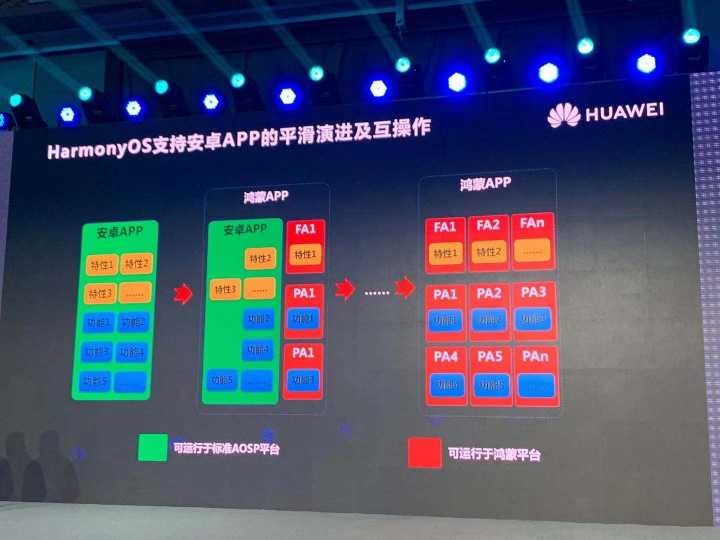刚才已经提到，当前的优酷鸿蒙专版包含Android APK主体，以及桌面Widget HAP， 多屏互动HAP。

因此，鸿蒙版优酷不仅拥有Android版优酷的的所有功能, 还拥有Android版不具备的一些特殊功能。

优酷鸿蒙版是在早期吃螃蟹,和华为一起合作开发鸿蒙版App先行者之一,解决了大量实际工程难题，并且与华为共同解决了大量开发环境和运行时的Bug，最终顺利地让优酷鸿蒙混合包上架华为应用商店。

打包方案Battle

分包方案

将原Android Apk应用和Harmony Hap（Hap为Harmony应用编译产物，跟Apk角色一致）应用分别上架应用市场。

• 优点：Apk和Hap可以不同包名，单独控制版本上架
• 缺点：用户需要同时下载安装 2次才可以体验完整功能

混合包方案

将原Android Apk和Harmony Hap混合打包成App上架应用市场。

• 优点：用户下载安装 1 次即可体验完整功能，不必担心2个应用版本差异
• 缺点：打包生产相对麻烦，对apk和hap有同包名限制

对比下，分包方案需要安装2次才可以完成整体功能体验，这显然是硬伤，合包方案虽然在开发生产侧麻烦一些，但可以给用户带来较好的体验，所以选择了混合包方案。

混合包

什么是混合包？

Android的产物是Apk，Harmony的产物是Hap，将Apk和Hap混合打成一个总产物包称作为混合包（APP）。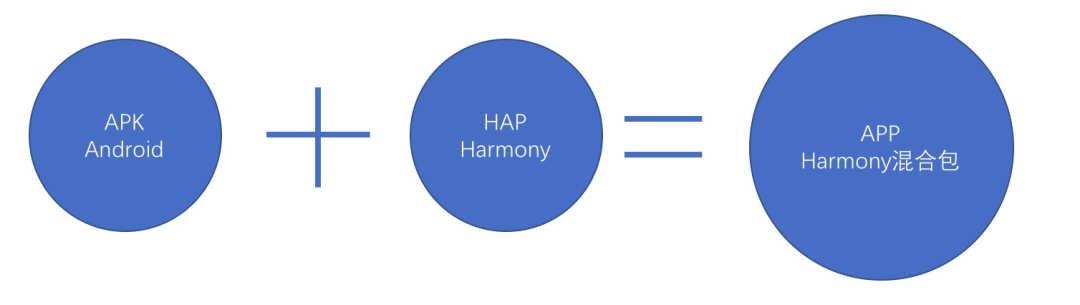混合包使用场景

场景1：当Harmony版应用并不是一个完整的功能，而只是作为原有Android应用的能力补充和增强时，需要将apk和hap打包成一个整应用。

场景2：Android应用短时间内无法全部迁移成Harmony版，分节奏迁移过程中可采用此方案进行混合打包作为一个供Harmony系统可运行的应用。

混合包打包流程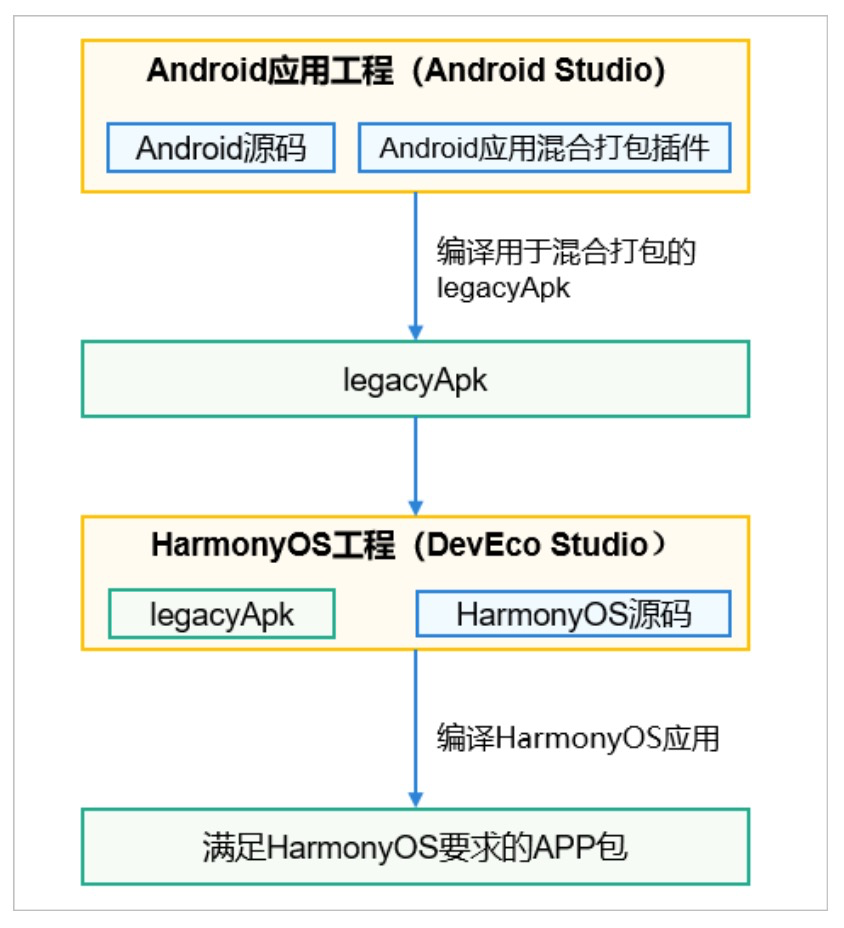名词解释：

• legacyApk：在原有正常Android工程和混合打包插件编译出的apk产物，此apk不能独立安装和运行，仅用于生产鸿蒙最终app的中间产物。如上图：混合打包流程实际就是将legacyApk（经过加工的原Android Apk产物）和鸿蒙应用产物Hap打包成一个App包（zip）的过程。

混合包要求及限制

名词解释：

• 鸿蒙entry：对应Android的application；
• 鸿蒙feature：对应Android的module或bundle。

限制：

• 鸿蒙工程entry中将作为apk的父容器，所以不能包含任何代码和资源，需将所有的代码和资源移到feature中；
• 鸿蒙entry和feature们的config.json中必须保持一致，并且与Android的versionCode versionName保持一致；
• Android应用（legacyApk）必须为64位包，不能包含32位的so。

要求：

• 鸿蒙应用必须与原Android应用同包名；
• 混合打包要求hap（类Android的agp）版本 >= 2.4.2.2，apiVersion（类Android的targetSdkVersion）需要>=5；
• 鸿蒙应用启动时实际还是单独应用，为了保持跟原Android技术品牌一致，需要保持entry中的config.json中的label和icon与原安卓应用中一致。

生成步骤

第一步：原Android Apk侧修改

为了干预原Apk的启动流程，增加鸿蒙应用的启动入口，需要干预Android的application。鸿蒙侧提供了工程侧打包编译的plugin，但由于优酷是组件化开发模式，application作为一个单独的aar模块存在，所以混合编译plugin并不是适用。

方案：通过了解混合编译plugin的职责，直接在application模块手动修改父类为AceHarmonyApplication（ohos.ace.ability.AceHarmonyApplication，此类在鸿蒙提供abilityshell.jar中），并手动在manifest中添加用于鸿蒙兼容性属性如下：

<!--鸿蒙兼容性属性-->
<uses-feature android:name="zidane.software.ability"
android:required="false" />
<meta-data android:name="permZA" android:value="true" />
<meta-data android:name="multiFrameworkBundle" android:value="true" />

将application模块集成进Android工程，编译后得到产物legacyApk。

第二步：鸿蒙工程侧修改

1、配置工程参数

config.json

• entry和所有feature中的config.json的apiVersion中的compatible和target改成5；
• entry和所有feature中添加originalName属性并保持跟bundleName一致；
• entry和所有feature中添加version，并保持子属性code等于Apk中的versionCode，子属性等于Apk中的versionName；
• entry和所有feature中的vendor保持一致，config.json中的code和name有要求，name推荐为三段式"a.b.c" Code值为a*1000000+b*1000+c。如Name为1.001.003，Code值为1001003。
{
“app”:{

"bundleName”:”包名”, //这是鸿蒙应用包名，混合包要求必须与Apk包名一致
"vendor”:”xxx”,//entry和feature中要一致
"version":{
"code":xxx,//对应Android VersionCode要求高于apk版本，并且根据name拼接而成
"name”:”xxx” //对应Android VersionName
},
"apiVersion":{
"compatible":5,//5才支持混合包
"target":5,
"releaseType":"Release"
}

}
}

2、在entry模块添加legacyApkOptions配置

apply plugin: 'com.huawei.ohos.hap'
ohos {
...
legacyApkOptions {
legacyApk"..\\..\\legacy_entry.apk" //指向legecyApk文件，并且必须以entry.apk结尾
legacyVersionCode "499" // 与 legacyApk 的 AndroidManifest.xml 中配置的android:versionCode 保持一致
legacyVersionName "9.15.2" // 与 legacyApk 的 AndroidManifest.xml 中配置的android:versionName 保持一致
}
}

3、entry修改

将entry中的所有代码和资源移到feature中（鸿蒙工程允许有一个entry，可以有多个feature）；添加一个空的Ability页面，并修改icon和label与原Android应用一致。

第三步：签名

签名是混合打包中最麻烦的一步，这也是鸿蒙开发最特殊的一步，需要拿原签名文件(jks或者p12)用DevEco Studio生成csr，然后去华为应用市场申请签名的证书（cer）文件和Profile（p7b）文件，更多详情也参考华为帮助文档（https://developer.huawei.com/consumer/cn/doc/distribution/app/agc-help-harmonyos-debugapp-0000001058642113）

由于混合包要求APP的签名信息要与原Android的签名信息一致，所以正常情况下用原Android的签名文件（jks）就可以了，但鸿蒙为了安全性，提升了签名的安全性要求：

1. 必须使用EC算法
2. 密钥密码要”大小写字母/数字/ 特殊字 符，至少两种的组合，长度大于等于 8

如果签名文件构建的时间比较早，这两个要求都不符合的话，华为侧提供了如下解决方案：

1、可以使用原Android签名文件单独配置shell Apk签名

apply plugin: 'com.huawei.ohos.hap'
ohos {
...
legacyApkOptions {
...
signConfig {
storeFile file("..\\legacyApkJks.jks") // 单独配置的 shell apk 签名材料
}
...
}
}

2、使用keytool命令行生成p12文件和csr文件，但要求别名和秘钥跟原Android签名保持一致，并且使用EC算法

//生成p12
keytool -genkey -alias [keyname] -keystore [p12.file] -storetype pkcs12 -keyalg EC -keypass [password] -storepass [password]

//生成csr
keytool -certreq -alias [keyname] -keystore [p12.file] -storetype pkcs12 -file [csr.file]

申请下来cer和p7b文件（需要单独申请调试和发布证书）后，就可以在开发工具中配置签名文件了（更多配置详情也阅览华为配置签名帮助文档 https://developer.harmonyos.com/cn/docs/documentation/doc-guides/publish_app-0000001053223745#ZH-CN_TOPIC_0000001058015911__section9752152162813）。

然后通过开发工具DevEco Studio中build -> Build Apps编译得到产物APP。

工程结构概述：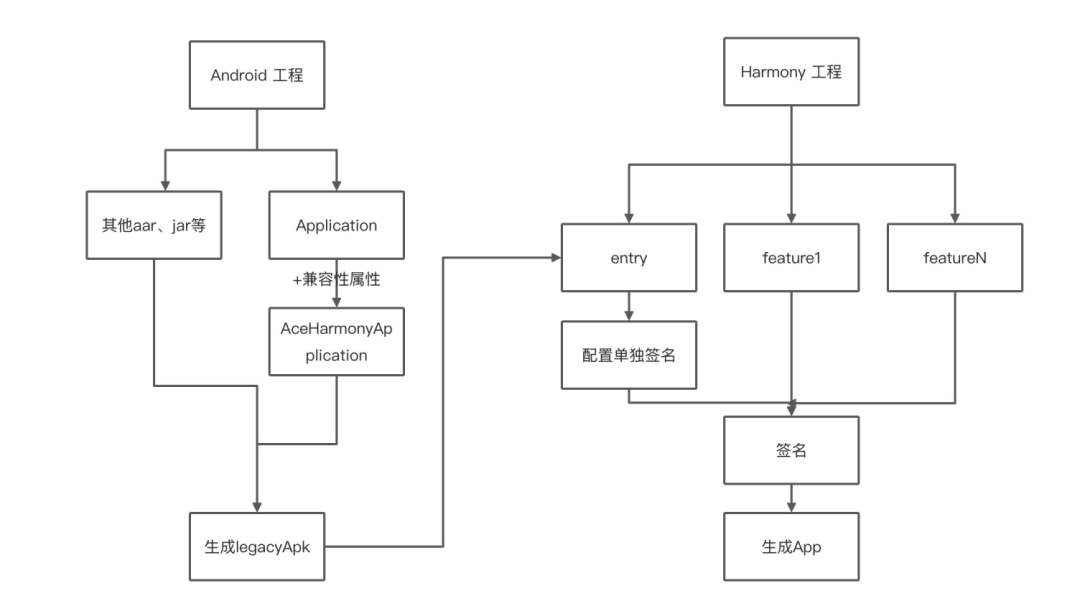混合包产物分析

经过上面的一系列流程，就可以生成一个供鸿蒙运行的混合包了。

由于是发布证书签名，这个混合包实际上是不能直接手动安装到Harmony OS上，还需要经过华为平台侧（需要联系华为侧接口人）签名，提供转换之后的安装包供优酷方面测试使用。

测试完毕之后，可以直接拿这个混合包上架到华为的鸿蒙应用市场。

鸿蒙版本发版经验总结

• 鸿蒙混合包只有64位包；
• 鸿蒙混合包在鸿蒙市场发版节奏、版本号等最好是跟原安卓发版节奏、版本号等保持一致，不然需要特殊考虑鸿蒙版本的数据定投问题；
• apk和hap在app中实际是物理区分，打包app的时候并不会把apk重新编译，所以如果apk和hap存在同名的类（因为都是java类），根据双亲委派机制，在运行时将会出现问题；
• 如果原安卓应用包含通讯录、拨打电话权限，在华为平台申请p7b文件时一定需要勾选申请使用受限权限，如下图：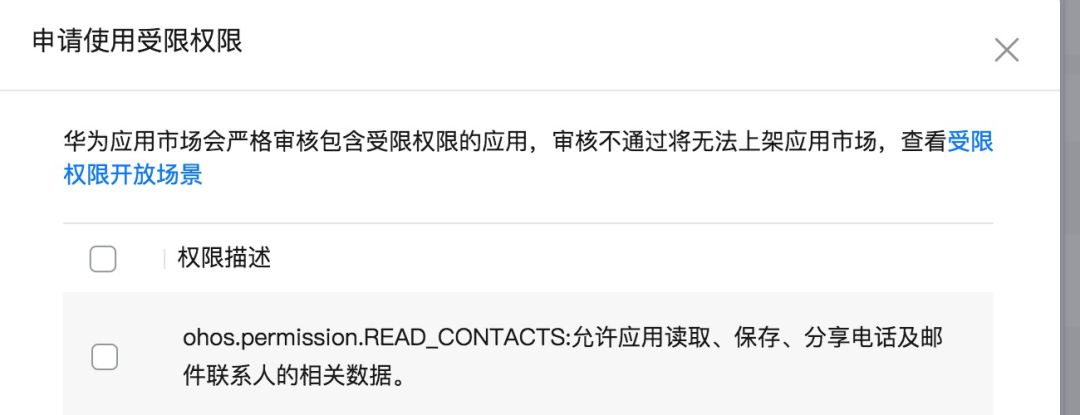最后，下周《优酷鸿蒙开发实践》系列第三篇技术文章，我们将以优酷播放中心的技术储备为切入点，结合鸿蒙系统的镜像和流转特性，详细介绍普通流转、自由视角和zoom 等核心能力在鸿蒙上的实践之路。

感谢关注【阿里巴巴移动技术】，我们下篇技术实践再见。

关注我们，每周 3 篇移动技术实践&干货给你思考！

展开全文harmonyos android 移动开发
• MySQL数据库管理与开发实践教程_14027075.pdfMySQL数据库管理与开发实践教程_14027075.pdfMySQL数据库管理与开发实践教程_14027075.pdf[General Information]书名=MySQL数据库管理与开发实践教程作者=程朝斌页数=395...

MySQL数据库管理与开发实践教程_14027075.pdfMySQL数据库管理与开发实践教程_14027075.pdfMySQL数据库管理与开发实践教程_14027075.pdf

[General Information]

书名=MySQL数据库管理与开发实践教程

作者=程朝斌

页数=395

SS号

DX号=

出版日期=2016.06

出版社=北京清华大学出版社

封面

书名

版权

前言

目录

第1章 MySQL入门知识

1.1 MySQL概述

1.1.1 MySQL发展历史

1.1.2 MySQL主要特性

1.1.3 MySQL适用场景

1.1.4 MySQL分支版本

1.2 MySQL与其他数据库的区别

1.2.1 功能比较

1.2.2 易用性比较

1.2.3 性能比较

1.2.4 可靠性比较

1.3 MySQL 5.6.1 9功能概述

1.4 实验指导——在Windows下安装MySQL

1.5 MySQL基本操作

1.5.1 启动和登录MySQL

1.5.2 常用命令

1.5.3 MySQL配置文件

1.6 实验指导——使用MySQL Workbench管理MySQL

1.7 MySQL实用工具

1.7.1 查看工具集

1.7.2 mysql工具

1.7.4 mysqlshow工具

1.7.5 mysqlbinlog工具

1.7.6 perror工具

思考与练习

第2章 MySQL数据库体系结构

2.1 MySQL文件结构

2.1.1 数据文件

2.1.2 日志文件

2.2 系统架构

2.2.1 架构结构图

2.2.2 模块交互流程

2.3 MySQL存储引擎

2.3.1 MySQL存储引擎简介

2.3.2 查看MySQL存储引擎

2.3.3 MyISAM存储引擎

2.3.4 InnoDB存储引擎

2.3.5 MEMORY存储引擎

2.3.6 其他存储引擎

2.3.7 如何选择存储引擎

2.4 实验指导——更改MySQL默认存储引擎

2.5 数据类型

2.5.1 整数类型

2.5.2 浮点类型

2.5.3 字符串类型

2.5.4 时间日期

2.5.5 集合类型

思考与练习

第3章 操作数据库和表

3.1 数据库和表概述

3.1.1 数据库概述

3.1.2 表概述

3.2 创建数据库

3.2.1 MySQL Workbench创建数据库

3.2.2 SQL语句创建数据库

3.3 管理数据库

3.3.1 查看数据库

3.3.2 修改数据库

3.3.3 删除数据库

3.4 创建数据表

3.4.1 MySQL Workbench创建数据表

3.4.2 使用数据表模板

3.4.3 SQL语句创建数据表

展开全文• 经过半年多的写作、修改、校样、印制，我们的实践教材《C语言课程设计与游戏开发实践教程》终于出版了。这本书可以看成是“做游戏，学编程（C语言）专栏”的详细版本，以下为书中部分案例的运行效果，本书所有案例均...

经过半年多的写作、修改、校样、印制，我们的实践教材《C语言课程设计与游戏开发实践教程》终于出版了。这本书可以看成是“做游戏，学编程（C语言）专栏”的详细版本，以下为书中部分案例的运行效果，本书所有案例均提供源代码下载。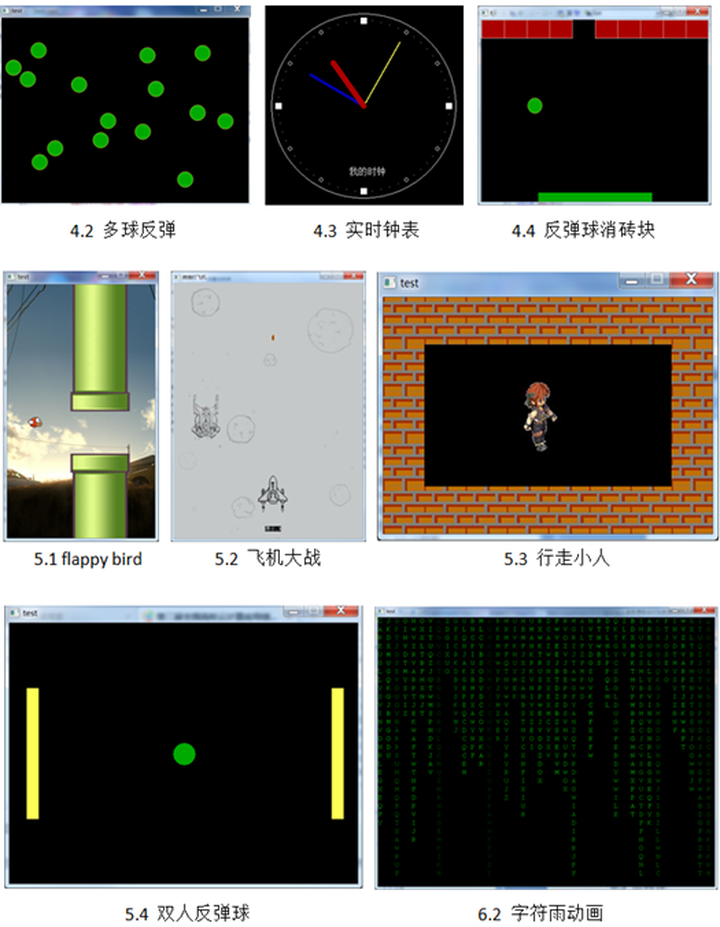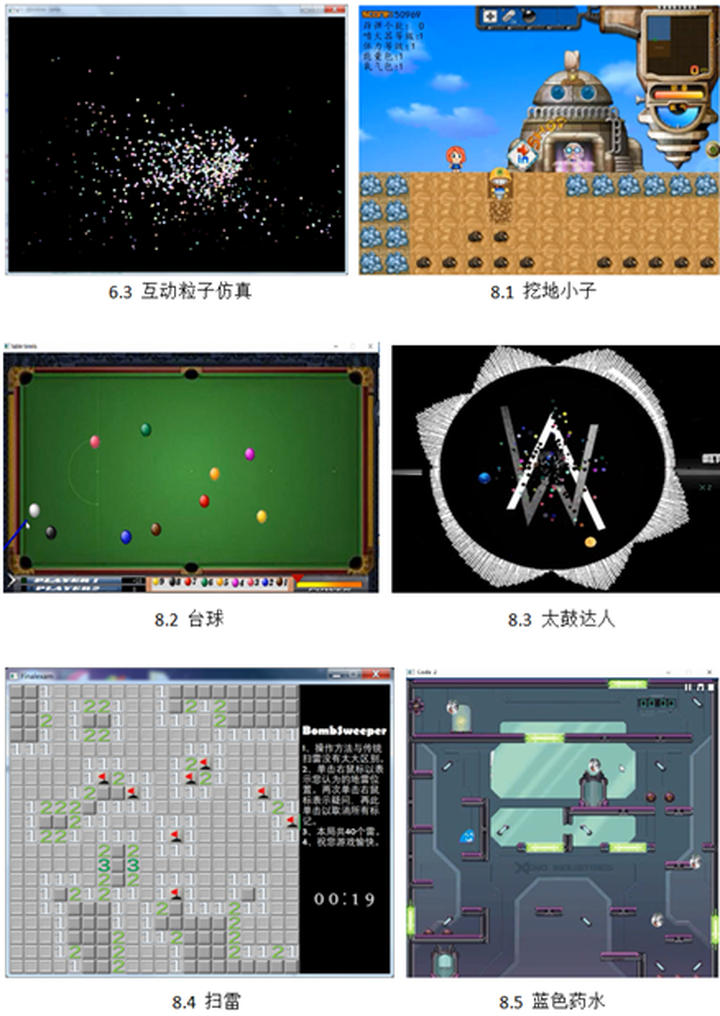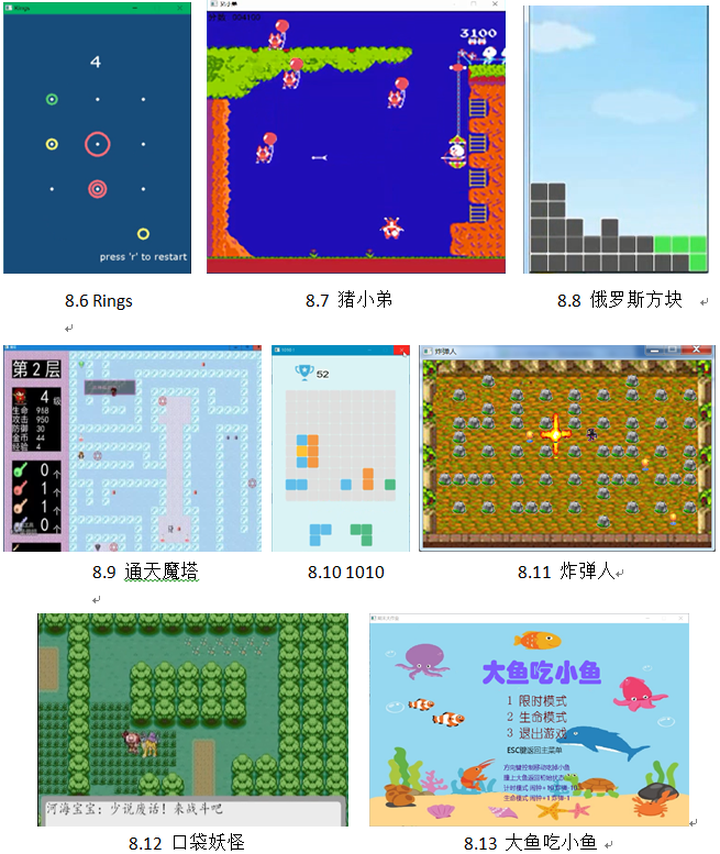图书前言：

C语言是一门重要的基础课程，应用广泛，也是不少后续课程的基础。然而，由于C语言语法规则较多，在实际编程时又相对灵活，很多初学者接触这门课程会觉得有难度，普遍有畏惧心理。目前配套教材一般偏向于语法规则的介绍，实例偏数学算法，过于抽象、趣味性不强，学生不愿写程序，进而觉得入门困难。

针对这些问题，本书把游戏开发实践应用于C语言课程设计教学，培养学生对编程的兴趣。为了达到这一目的，本书应用C语言的语法知识带领同学从无到有地开发游戏，通过游戏案例逐步将已学的语法知识用起来，在实际编程中加深体会。在设计的过程中尽量站在初学者的角度，降低编写游戏的难度，不超出所学知识范围，逐步提高读者对编程的兴趣和能力。

美国著名教育家杜威曾经说过：“大多数的人，只知道对五官接触的、能够实用的东西才有趣味，书本上的趣味是没有的”。同样对于C语言这门课程，让学生看到用C语言可以编出很好玩的程序，学生感到有趣、有成就感，就会自己花时间钻研，师生积极互动，教学效果也因此得到改进。

各章节主要内容如下：

第1章，学习printf、scanf、if-else、while、for语句后，进行弹跳小球、飞机游戏的开发，并介绍程序调试的方法与技巧。

第2章，学习函数后，利用函数封装及标准的游戏框架，进行飞机游戏、反弹球消方块、flappy bird的开发。

第3章，学习数组后，利用数组改进数据结构，实现生命游戏、反弹球消砖块、空战游戏、贪吃蛇的开发，并介绍SVN代码管理工具。

第4章，学习简单绘图工具，并进行多球反弹、实时钟表、反弹球消方块、鼠标交互的学习开发。

第5章，学习图片音乐素材的导入和使用，并进行flappy bird、飞机大战、行走小人、双人反弹球的开发学习。

第6章，利用后续语法知识进一步改进游戏程序，如指针创建动态数组、字符串控制得分显示、结构体改进数据结构、文件用于游戏存档等。实现了黑客帝国字符雨动画、互动粒子仿真、具有多界面和存档功能的飞机大战游戏。

第7章，利用游戏化学习的思路，学习C语言的两个知识难点：递归与链表。

第8章，介绍多个游戏开发实践案例：挖地小子、台球、太鼓达人、扫雷、蓝色药水、Rings、猪小弟、俄罗斯方块、通天魔塔、1010、炸弹人、口袋妖怪、大鱼吃小鱼。每个案例讲解了主体功能、实现思路。

图书的详细目录：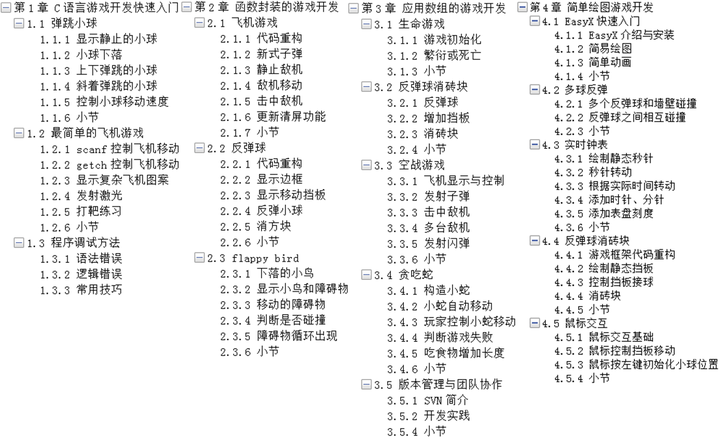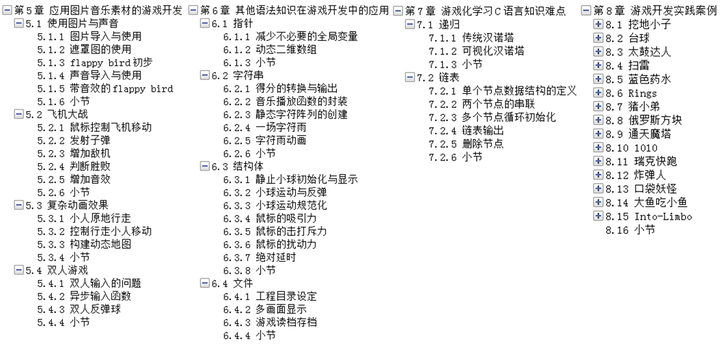本书的使用方法：

本书通过一个一个的游戏案例进行讲解，并按照C语言的学习进度，逐步使用更多的语法知识，难度逐渐加深。在每章内容开始前，会介绍学习该章所需的语法知识，大家在掌握对应语法后可以进入相应游戏案例的开发。每个案例会分成很多步骤，从零开始step by step地来实现，书中列出了每个步骤的实现目标、实现思路、相应的参考代码。读者可以先在前一个步骤代码的基础上，尝试实现下一个步骤，碰到困难再参考书中给出的例子代码。在每个案例讲解后列出了一些思考题，读者可以尝试进一步改进。

本书不讲解C语言的基础语法知识，读者可以通过相应入门教材、在线慕课进行学习，并配合Online Judge进行练习。

书中游戏案例开发使用操作系统为Windows，缺省开发环境为Visual C++ 6，也可以使用高版本的Microsoft Visual Studio进行开发。

-----------------------

图书的其他信息可以参考清华大学出版社上的介绍：清华大学出版社-图书详情-《C语言课程设计与游戏开发实践教程》

图书附带的源代码可从百度网盘下载：http://pan.baidu.com/s/1skGKbj3

希望这本图书能对C语言初学者有所帮助，也欢迎大家多提宝贵意见，谢谢！

展开全文编程语言 c语言 java python
• 文章目录SpringBoot-SSE开发实践SSE开发实践项目框架项目依赖Session管理业务接口业务实现任务异常控制器测试 SpringBoot-SSE开发实践 SSE SSE（Server-SentEvents，即服务器发送事件）是围绕只读Comet交互推出的API...java
• 京东鸿蒙版来了〜背景随着鸿蒙2.0的发布，华为部分手机用户迎来鸿蒙时代，京东作为华为鸿蒙OS的合作APP，首次投入鸿蒙应用商用版开发，目前已上架V10.0.2版本。鸿蒙OS特性2021年6...人工智能 java android 大数据 编程语言
• 软件工程案例教程答案（第三版）韩万江 姜立新 编著 （软件项目开发实践）知识点总结第一章知识点第二章知识点 从本学期开始，整理每一门课的笔记，学完一章一总结~~ (◕ᴗ◕✿) 写的不对的地方欢迎指正 课后习题...
• 软件危机：计算机软件开发和维护过程中遇到的所遇到的一系列严重问题 软件危机主要表现 1.对于软件开发成本和进度的估计常常不准确 2.开发的软件不能满足用户要求 3.软件产品的质量低 4.开发的软件可维护性差 5.软件...软件开发
• 为广大开发者提供基于互联网技术栈的操作系统平台，极大简化了物联网 App 开发难度，提高开发效率。通过爱智云，EdgerOS 为开发者提供了强大的云-边-端协同能力，开发者无需关心设备是本地还是远程连接，EdgerOS ...
• 前端组件化开发实践总结

千次阅读 多人点赞 2021-10-13 12:46:52
自从 2010 年第一份工作接触了前后端半分离的开发方式之后，在后面的这些年里，对前端的组件化开发有了更全面一点的认识，组件化在我们的前端开发中，对提高开发效率、代码的可维护性和可复用性有很大帮助，甚至对跟...java html vue.js 前端 组件化
• Nginx，从基本原理到开发实践（二）

千次阅读 多人点赞 2021-07-10 16:20:56
文章目录一、前言二、Nginx实现Http缓存2.1 理论2.2 实践2.3 第三方插件：ngx_cache_purge三、Nginx实现动静分离3.1 静态资源和动态资源3.2 实践四、Nginx实现数据压缩4.1 理论4.2 实践五、Nginx实现跨域访问5.1 同...nginx tomcat 运维
• Nginx，从基本原理到开发实践

千次阅读 多人点赞 2021-07-10 08:42:33
定义nginx的请求方式 4.2.3 proxy_set_header参数，取到客户端的网络信息 4.2.4 location参数，不同匹配规则和优先级 4.3 Nignx实现负载均衡，upstream参数 4.4 Nginx三大功能一起小结 五、实践——Nginx安装使用...java 开发语言 后端
• CodeHub#4：在线教育应用的开发实践 【主讲人】「荷小鱼」的前端工程师 雷文伟 【时间】2021/3/18 晚上 7 点 - 8 点 【地址】CodeHub 线上直播间 旨在解决的问题 面对市场快速发展进程中带来的用户下沉效应，网络...开发框架 移动开发 前端开发 大数据
• 作者简介Deway，携程资深工程师，iOS客户端开发，热衷于大前端和动态化技术；Frank，携程高级工程师，关注移动端热门技术，安卓客户端开发。前言随着各种多端技术的蓬勃发展，如今的移动...android java 移动开发 安卓
• DDS协议解读及测试开发实践

千次阅读 2020-12-23 15:43:18
DDS实现的数据共享可以理解成一个抽象的“全局数据空间”，任何应用程序，不论开发语言，或者运行的操作系统类型，都可以通过相同的方式访问这个“全局数据空间”，就好像访问本地的存储空间一样。当然“全局数据...中间件 经验分享 测试类型 编程语言 api
• 18_TCP服务器端代码案例 一、TCP服务器端代码实例 二、源代码 #1....server_socket = socket(AF_INET,SOCK_STREAM) #2....host_port = ('',8088) server_socket.bind(host_port) ...#3....server_socket.listen(5) #5:表示可以...网络 tcp/ip python
• Android AR开发系统文章之一：AR介绍 一、什么是AR 二、AR的发展历程 三、AR的应用场景 四、AR技术面临的主要问题 五、AR平台简介 一、什么是AR AR(Augmented Reality增强现实的简称），也有对应VR虚拟实境一词的...
• 在过去的一年中，经过跟支付宝移动端团队和广大开发者的交流和沟通，我们了解到大家在涉及到关于移动应用跨端开发的过程中遇到的一些问题，同时也在思考，我们可以对外输出那些行之有效的技术实践方案？开发团队在...
• ES作为一个开源的高扩展的分布式全文检索引擎，自2016年起已经超过Solr，成为排名第一的搜索引擎应用，具有很多独特的优点。
• SOME/IP相关技术干货及测试实践，也分享了1000BASE-T1物理层PMA测试相关测试实践，本期给大家介绍的是DoIP及以太网诊断测试开发相关知识及测试实践分享。 DoIP简介 以太网最早由BMW引入车内，其应用场景就是刷写，...编程语言 ethernet 车载以太网 DoIP
• 网络安全：关于SecOC及测试开发实践简介

千次阅读 热门讨论 2021-02-10 12:14:17
解决了仿真环境后，需要依据所开发的测试用例实现测试脚本。一方面验证正向的SecOC流程，另一方面验证SecOC机制的防“攻击”特性。通过使用CANoe的各个内置函数及外部第三方编程接口，对仿真条件进行相应的输入控制...网络安全 SecOC 经验分享 车载以太网
• 文章目录编写要求安装如何编写规则变量设置规则行为支持协议源信息方向操作符示例实践：编写规则完 编写要求 snort的安装与配置 自己设计snort规则发现入侵企图：1、入侵http服务或者ftp服务的入侵企图；2、使用...后端 linux snort 运维 网络协议
• 问题： moveit.1.1.2版的moveit设置助手生成的功能包，如果直接运行 launch XXXXX(包名) demo.launch 时会报错： Resource not found: moveit_resources_prbt_moveit_config ROS path =/opt/ros/noetic/share/...ubuntu
• 上篇文章“聊聊网络安全的5W1H”对网络安全知识体系和技术脉络做了深入浅出的介绍，提到AUTOSAR所定义的网络和通信安全相关的技术，本期我们将介绍其中的E2E策略（严格来说属于Safety的...，并分享在项目中的测试实践...网络安全 autosar E2E 测试
• 项目场景： 本周将要通过Qt和Opencv编写简单图片处理程序，主要实现图片的上传显示，对图片进行灰度化、二值化处理并保存。 问题与解决： 主要遇到了如下问题： 问题：ui中的成员没有更新，仍旧报错。...qt 图像处理 opencv
• 分享嘉宾：仰宗强编辑整理：刘春龙出品平台：DataFunTalk导读：本次分享嘉宾是来自贝壳大数据部门的仰宗强，详细介绍了针对贝壳的业务数据与需求的增长，逐步升级数据开发平台的探索实践过程...大数据 编程语言 java 数据库 分布式
• 《Unity AR增强现实开发实战》以Unity2018版本为开发平台，从增强现实的基本概念出发，系统介绍AR相关理论、行业应用及发展趋势，并且结合大量增强现实技术应用开发案例，从实战角度系统地介绍增强现实开发相关知识...
• 点击上方蓝字关注我们基于大数据的软件智能化开发方法与环境谢冰1,彭鑫2,3,尹刚4,5,李宣东6,魏峻7,8,孙海龙9,101北京大学信息科学技术学院，北京 1008712...大数据 微软 编程语言 devops 人工智能
• 用浏览器访问网站时，页面各不相同，你有没有想过它为何会呈现这个样子呢？本节中，我们就来了解一下网页的基本组成、结构和节点等内容。1. 网页的组成网页可以分为三大部分...下面我们分别来介绍一下这三部分的功能。...
• pip安装 目前，Beautiful Soup的最新版本是4.x版本，之前的版本已经停止开发了。这里推荐使用pip来安装，安装命令如下： 1 pip3 install beautifulsoup4 命令执行完毕之后即可完成安装。 4. wheel安装 然后使用pip......